Course EPS 109

Computer Simulations with Jupyter Notebooks

 Instructor: Burkhard Militzer GSI: Jizhou Wu militzer@berkeley.edu wjz8597@berkeley.edu Office hours: Tue 6-7 pm Tue 6-9 pm Online Online

 4 units: Lectures: Tu 5-6 pm Syncronous online Computer lab: We 9-11, 11-1, 2-4, or 4-6 Th 5-6 pm Syncronous online Syncronous online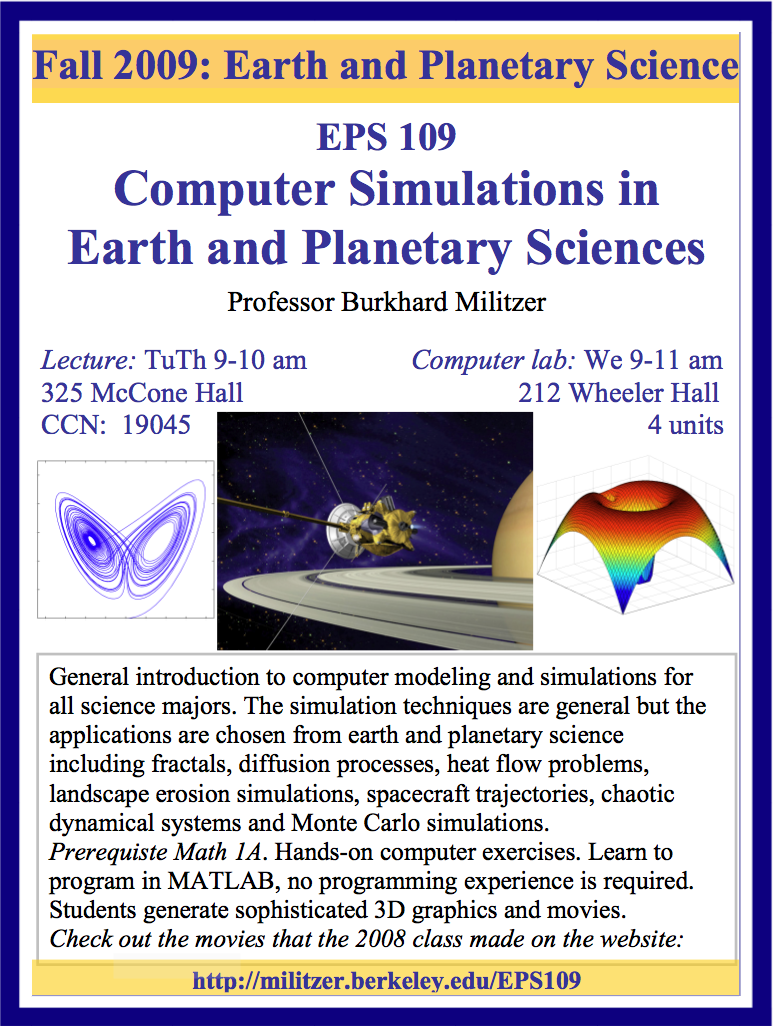This course offers a general introduction to computer simulations for all science majors. Participants will use the Jupyter notebooks to perform calculations, design 3D graphics, and build on existing computer programs. No prior programming experience is required. The course teaches fundamental skills for scientists and helps students with future homework. It covers data processing techniques as well standard simulations methods including Monte Carlo and molecular dynamics. The algorithms are illustrated with a variety of applications in earth and planetary science as well as astronomy.

Check out the animations made by students of our fall 2008, 2009, 2011, 2012, 2013, 2014, 2015, 2016, 2018, 2019, and 2020 classes.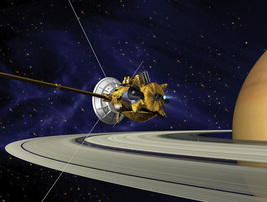Cassini spacecraft in Saturn orbit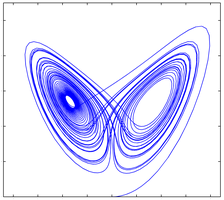Choatic behavior of dynamical systems: the Lorenz attractor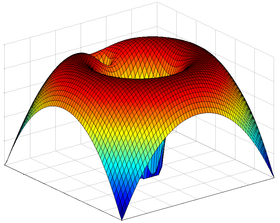Combined gravity field of sun and planet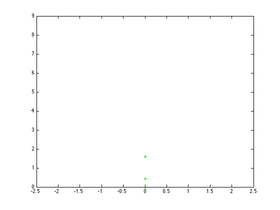Fractal nature of fernsKoch snowflake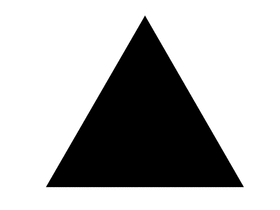Sierpinski gasket

Selected computer lab exercises and homework assignments from our 2018 Python class:

In 2018, the class switched from using Matlab to Jupyter notebooks. While there appears to be a general trend in that direction, the real motivation was the fact that all our students' Matlab codes would stop working once they left the university and their relatively inexpensive Matlab student licenses would expire. To do the following Jupyter exercises, we recommend

1) you install Anaconda on your Mac or PC.
2) Make a new directory Jupyter in home directory and place any data files like ice_core_temperature_data.txt there.
3) Start the Jupyter system from a Terminal with the commands:
cd ~/Jupyter
jupyter lab
4) Start a new notebook "File->New notebook" and step-by-step paste the commands for the first lab assignment Intro_to_python_05.pdf. Press SHIFT+RETURN each time.

 Type of assignment Description Files Lab 1 Getting to know Jupyter notebooks Intro_to_python_05.pdfice_core_temperature_data.txt Homework 1 Simple calculations with Jupyter notebooks hw01_course_intro13.pdfice_core_CO2_data.txt Lab 2 Pixel graphs and filling matrices pixel_graphics12.pdftopography_180x360_grid.txt Homework 2 Practice making a smiley hw02_pixel_graphics_13.pdf
In this course, we generate a number of simple animations and store them as MP4 files. Here are two examples wave1d.ipynb and wave2d.ipynb that use the FFMPEG package in combination with the screen grab function. We found them more convenient than typical Python animations packages that require the user to supply the graphics for an arbitrary frame index.

Selected computer lab exercises and homework assignments from our 2008 Matlab class:

 Type of assignment Description Files Lab 1 Getting to know Matlab: vectors & matrices lab01_Matlab_intro02.pdf Homework 1 Getting to know Matlab: loops and plots hw01_plotting_and_loops.pdf Lab 2 Fractals in Matlab: Mandelbrot set lab02_Mandelbrot01.pdfexample.mmandelbrot14.m Homework 2 Fractals in Matlab: Julia set hw02_Mandelbrot_and_Julia_sets.pdf Lab+homework 3 Diffusion limited aggregation lab_hw03_DLA_html.pdfdiffusion_limited_aggregation2D_exercise.m Lab 4 Keplerian orbits of planets integrated with Euler's method lab04_kepler04.pdfrun_kepler_ode_euler04.m Homework 11 Cooling of a lava dike hw11_cooling_dike.pdf

Additional examples that will be discussed in this course:Tadpole orbitHorseshoe orbit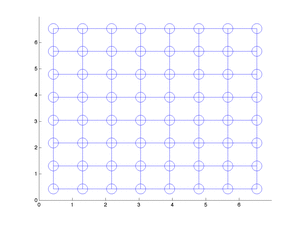Molecular dynamics simulations using a Lennard-Jones pair potential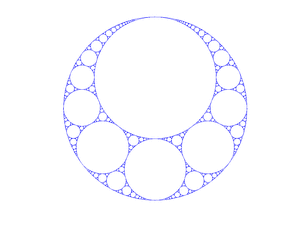Apollonian gasket Protect yourself from lighting with a Faraday's cage by solving the Laplace equation ;=) Dynamical simulation of the Lorenz equationDiffusion limited aggregation along a sticky wallModified aggregation to increase the fractal dimensionModified diffusion limited aggregation to grow more compact structures. Click on the images to start an animation.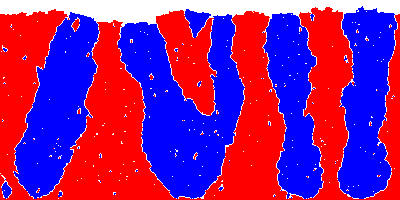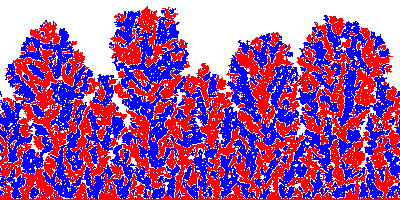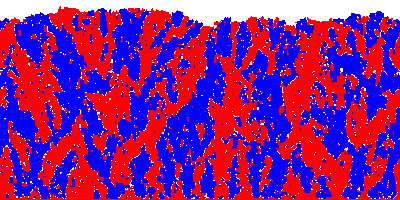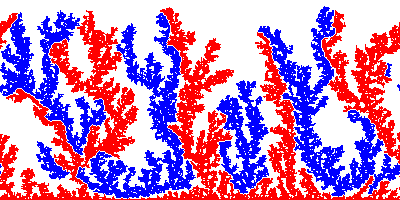Diffusion limited aggregation with two species. Click on each image to see the structures growing.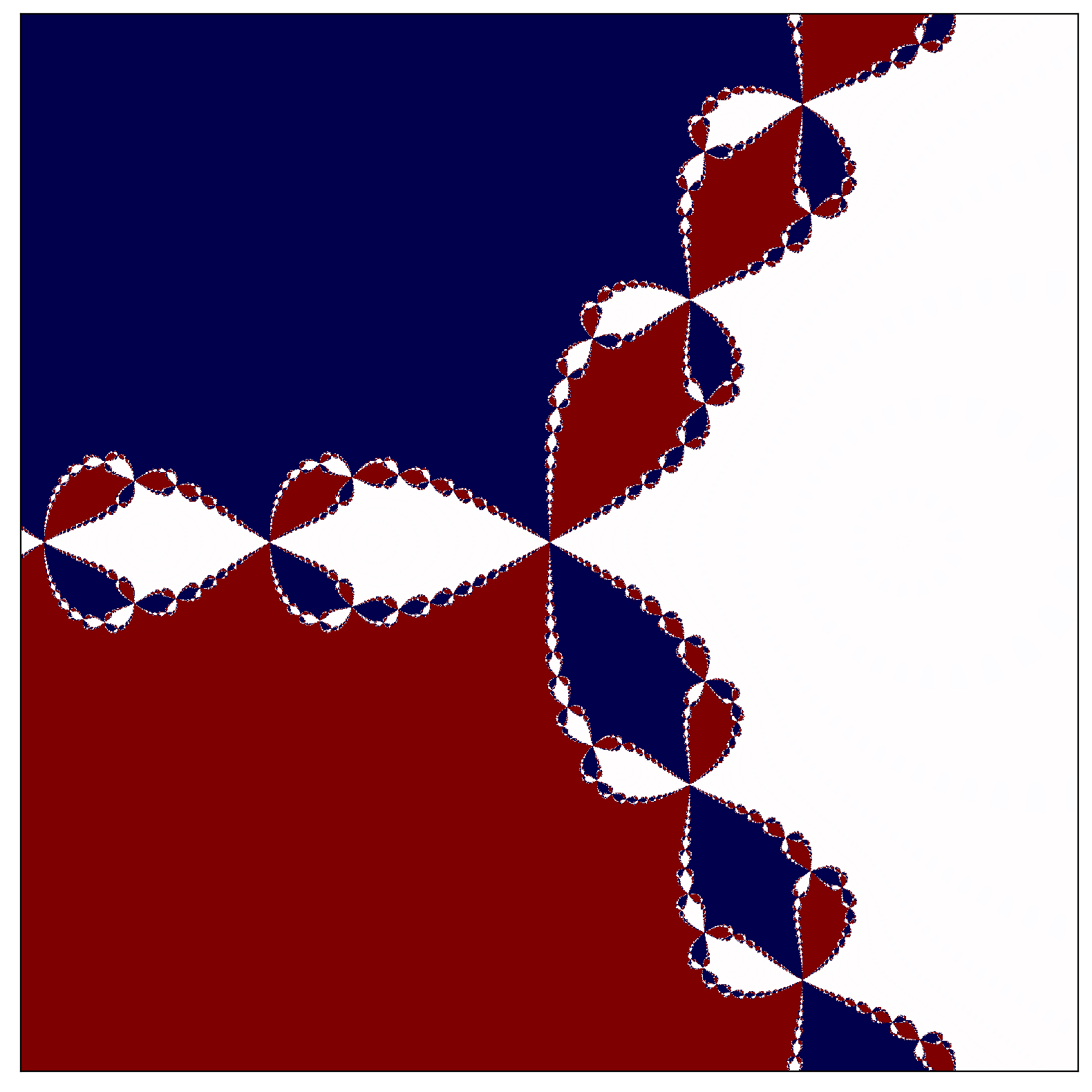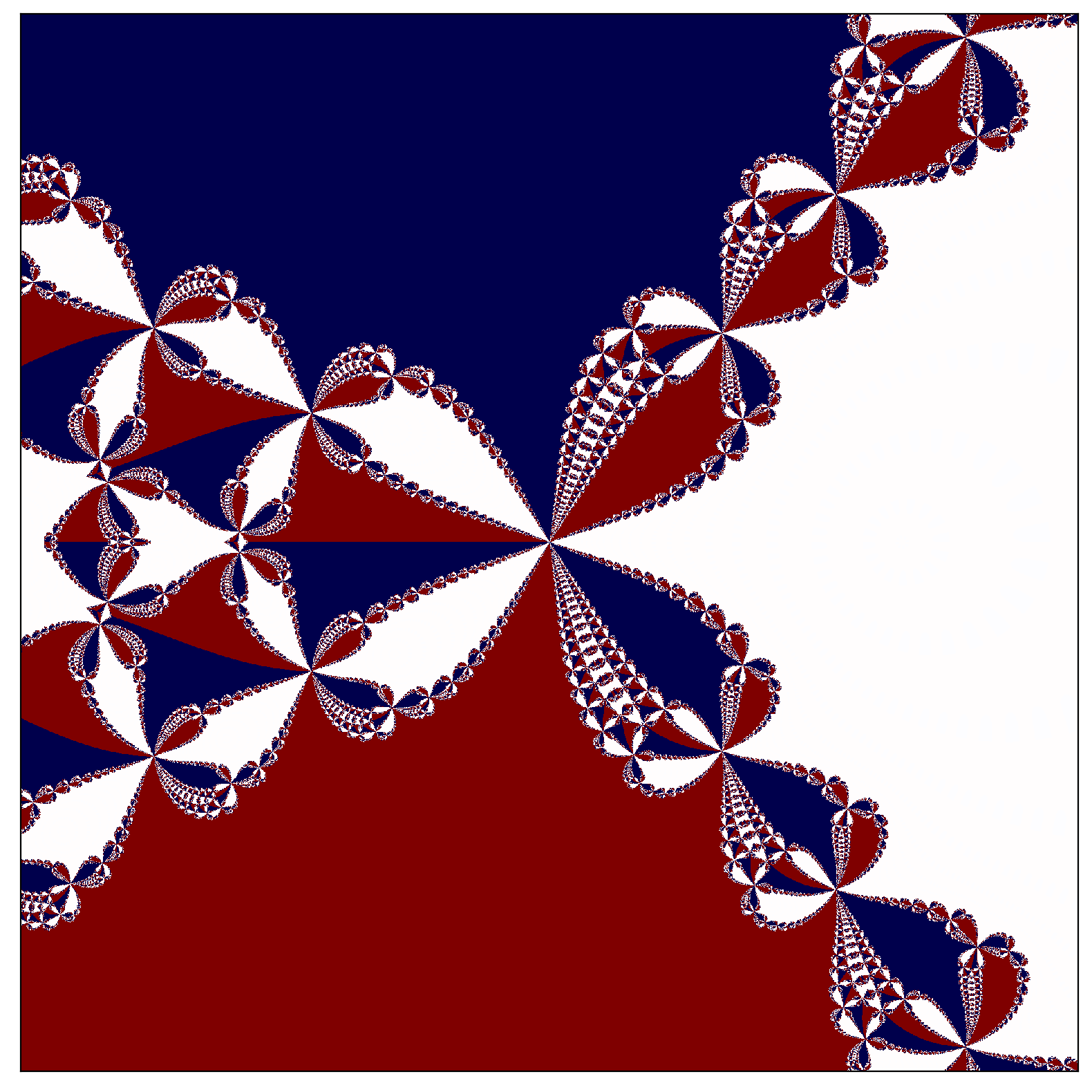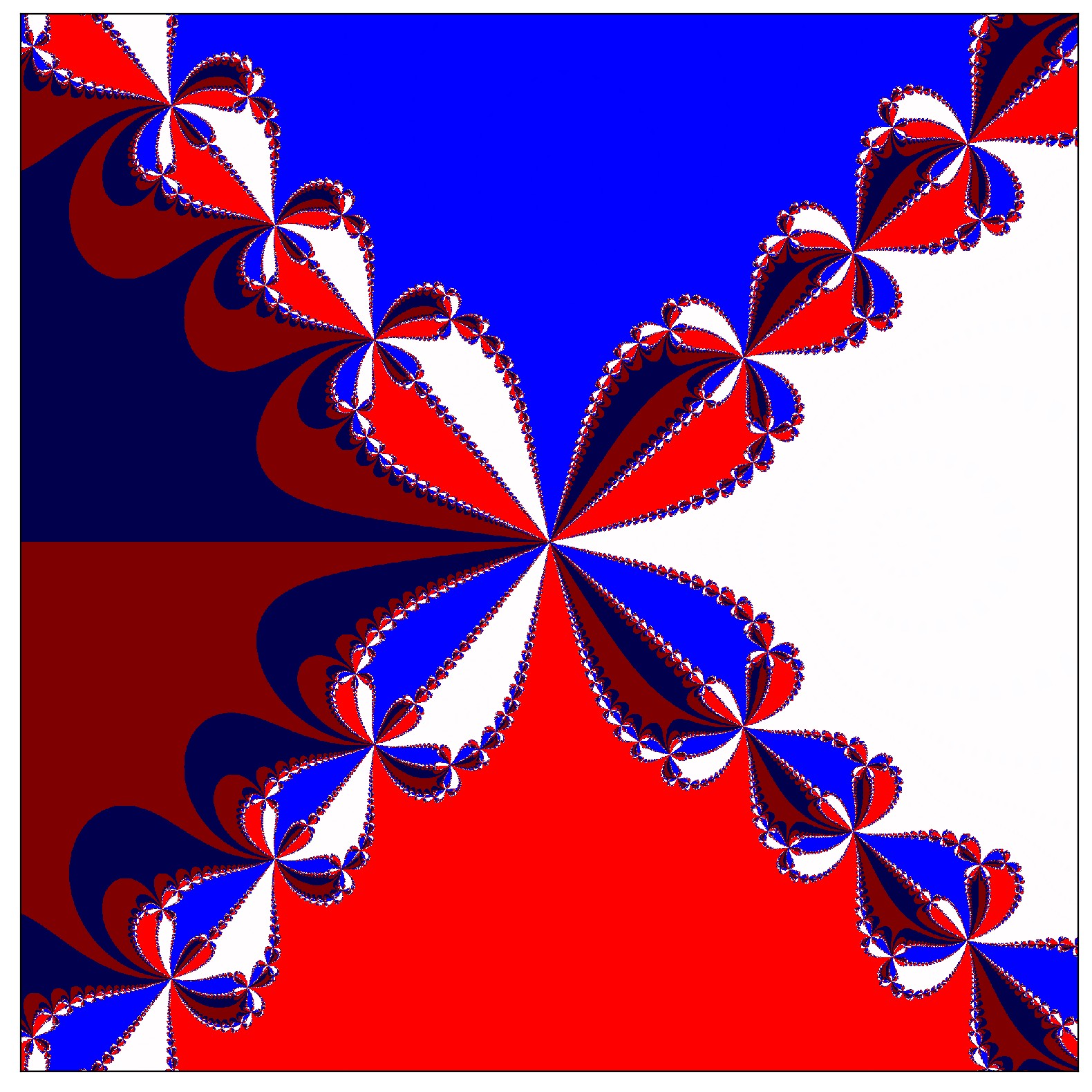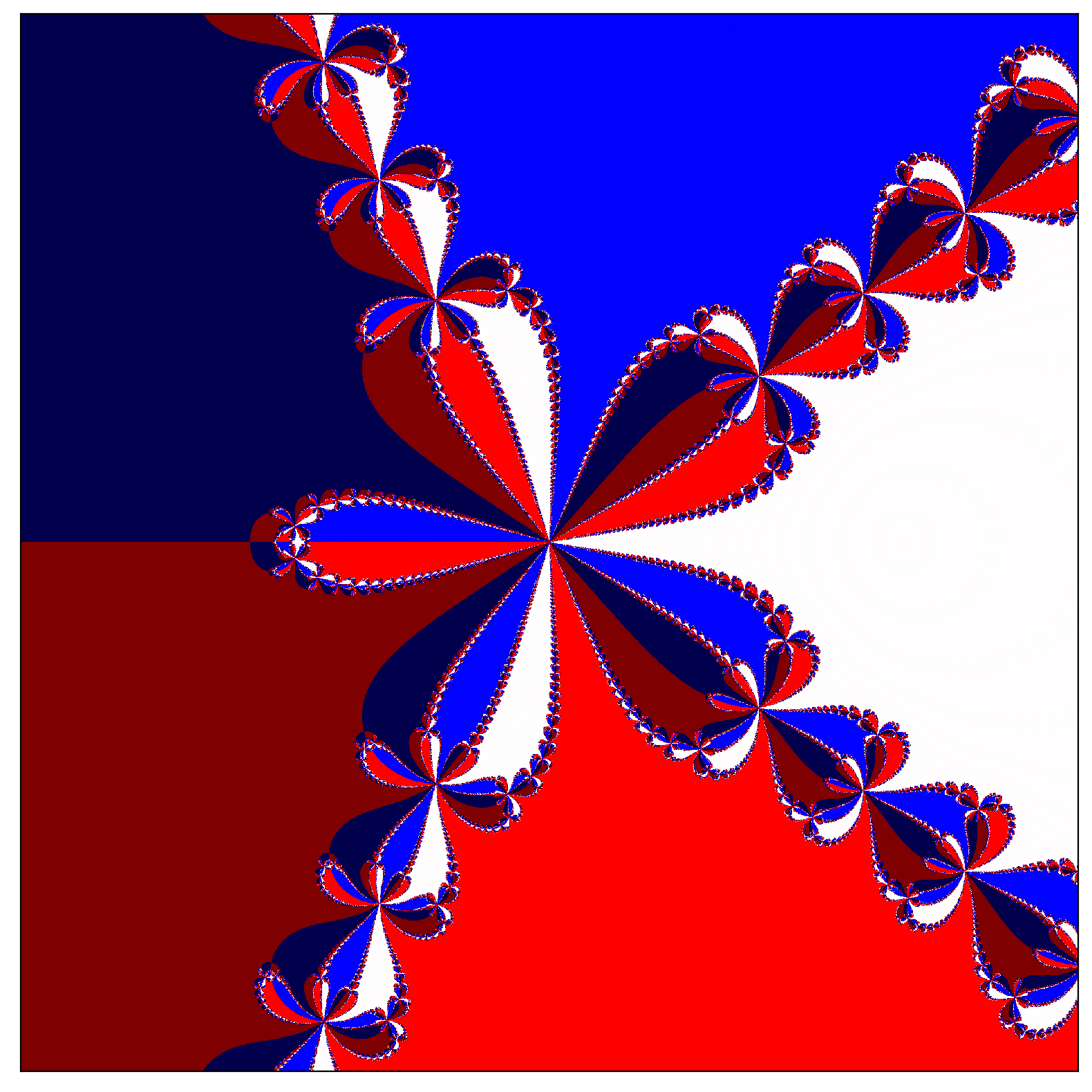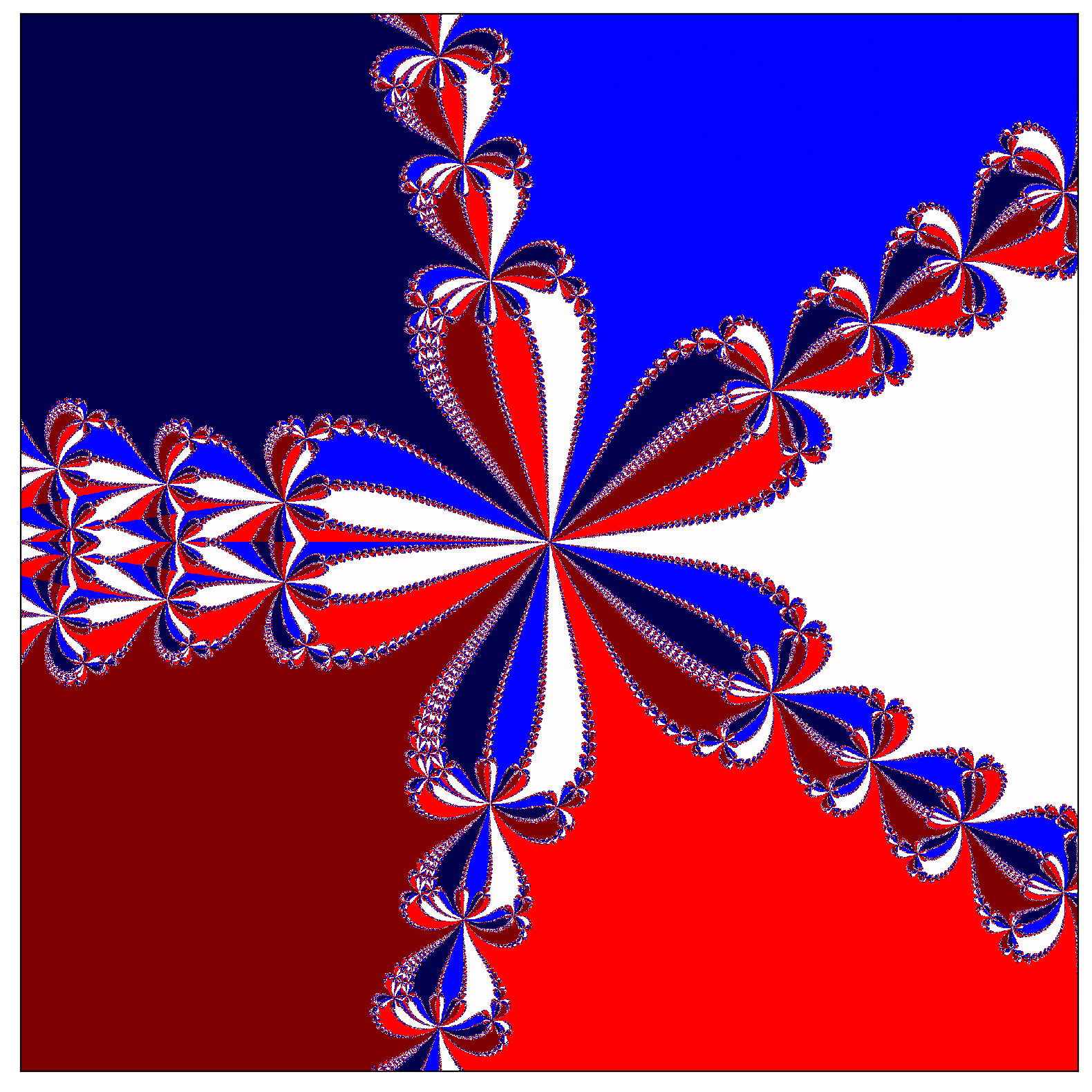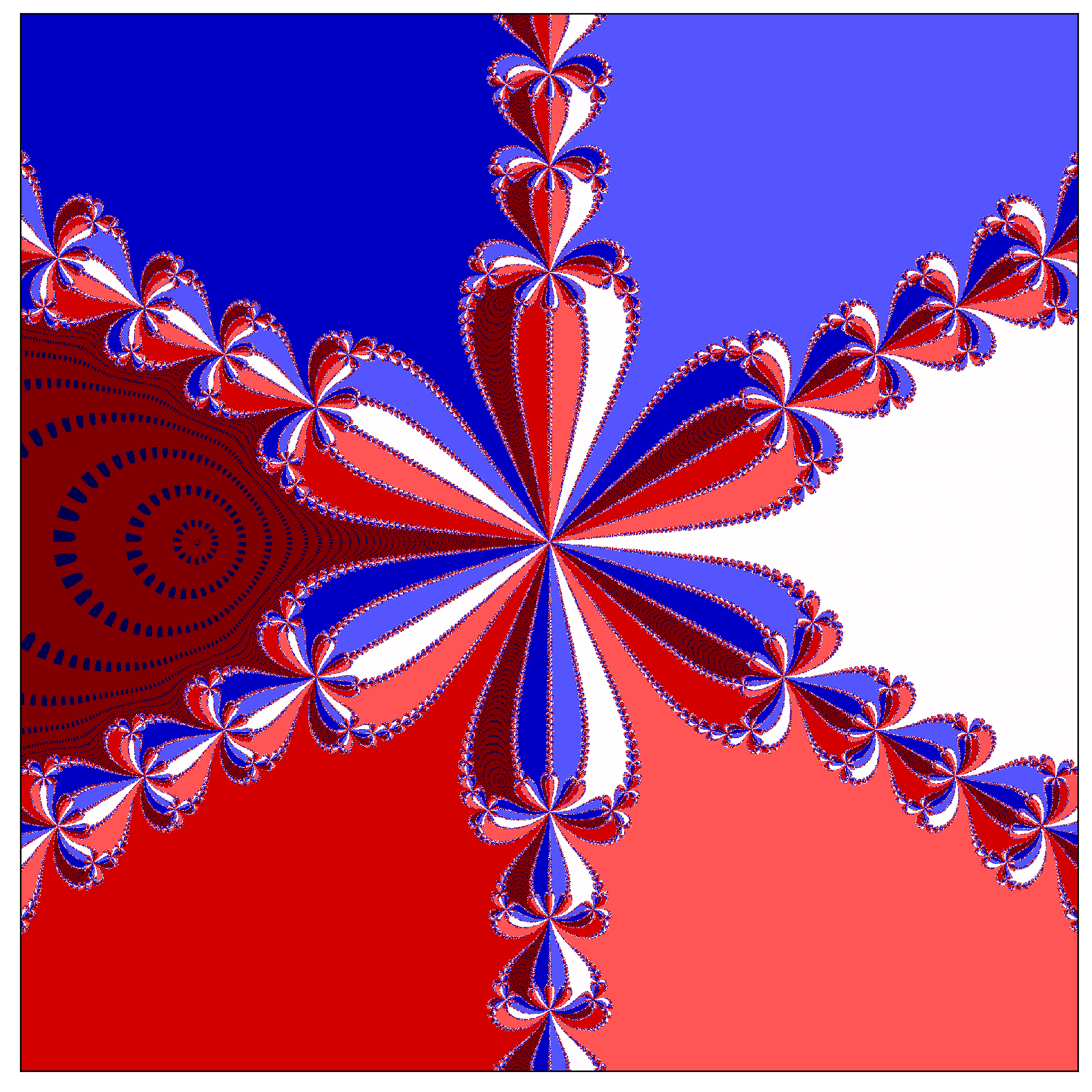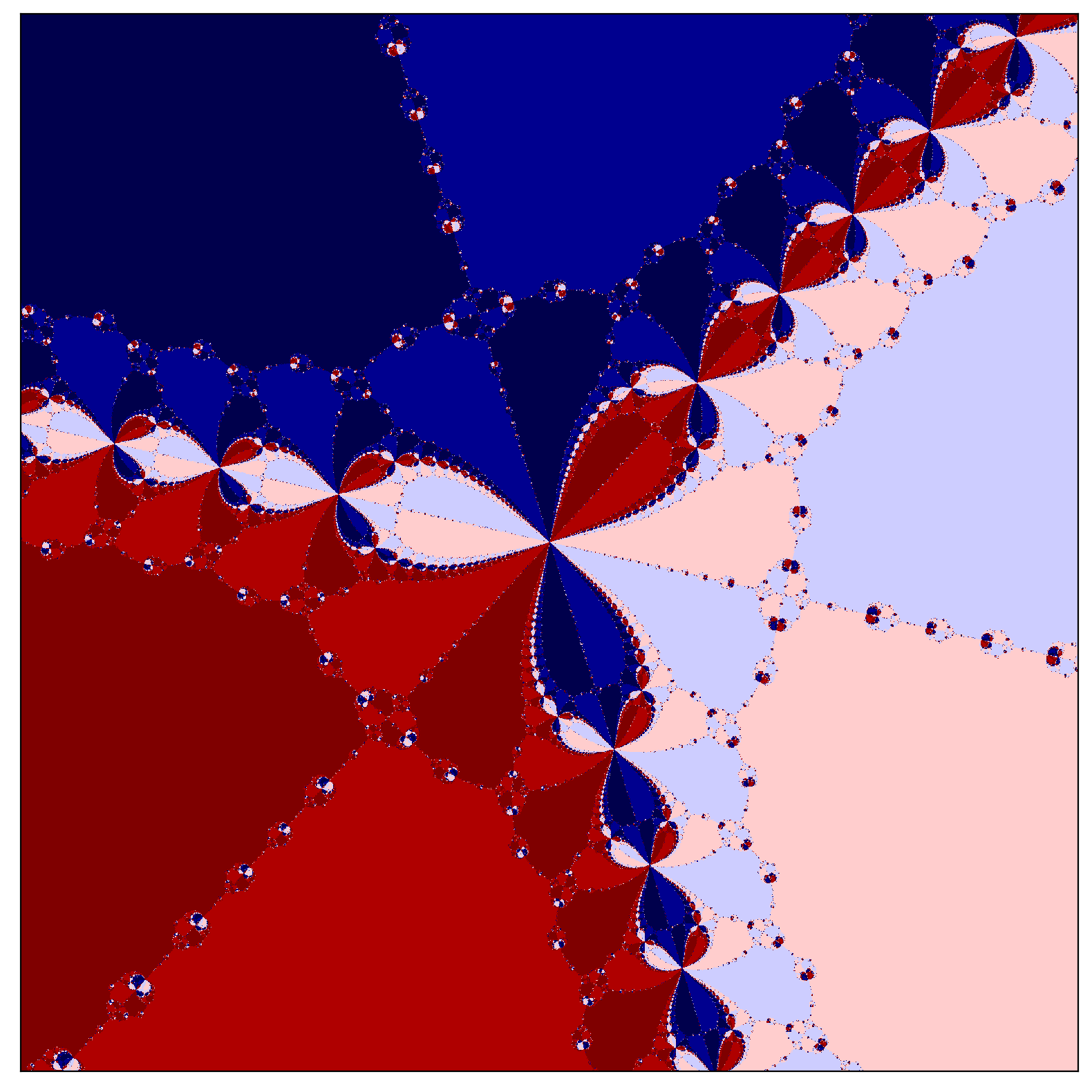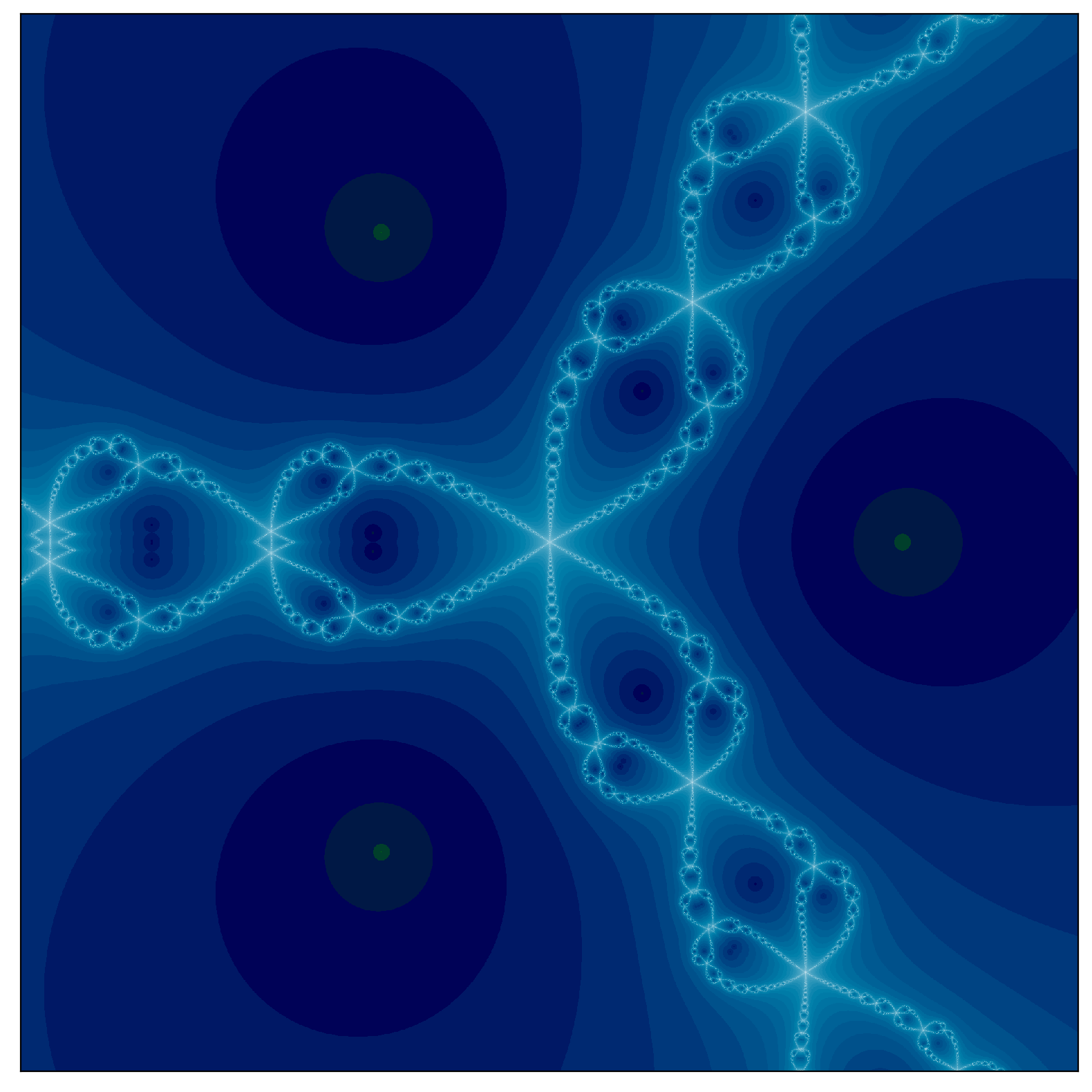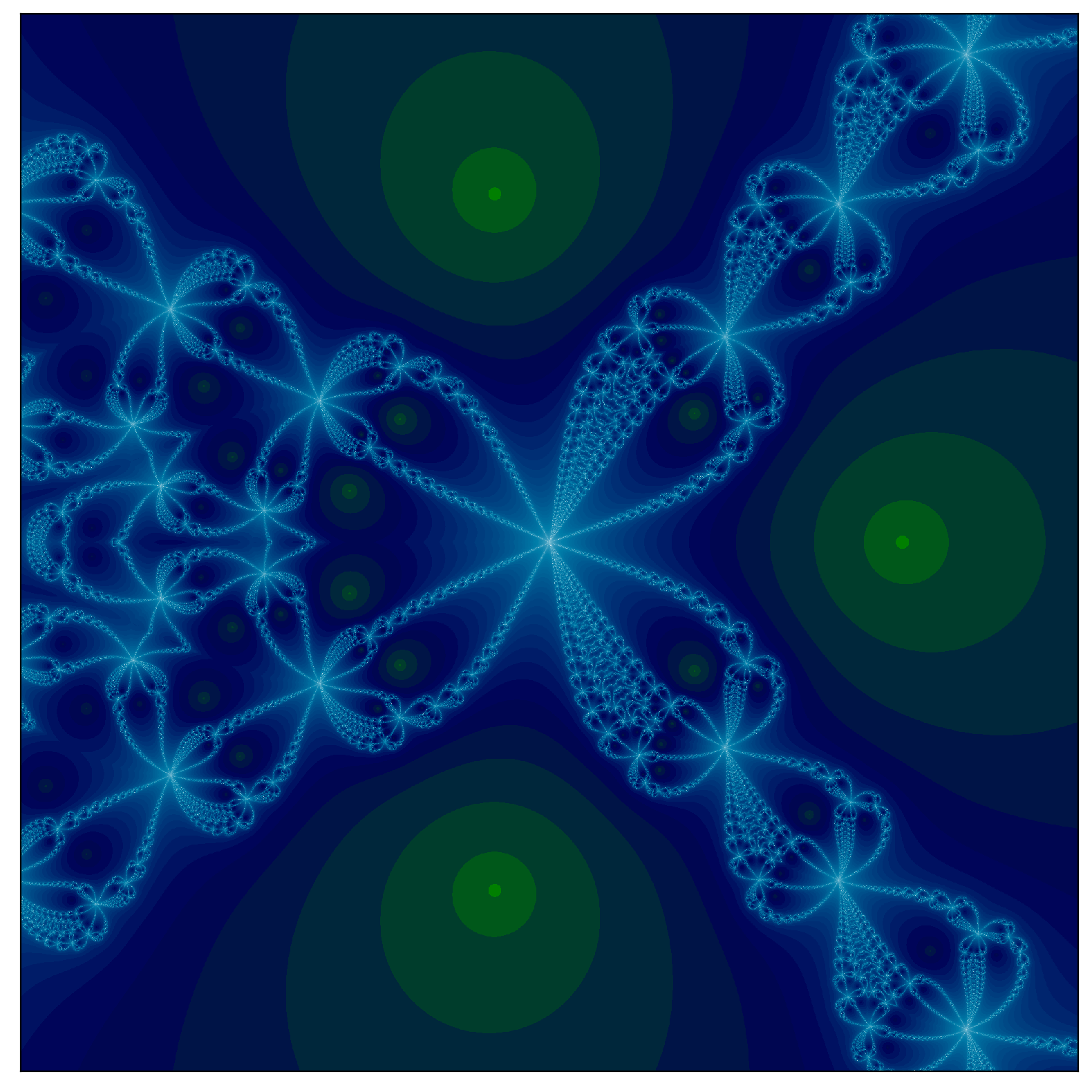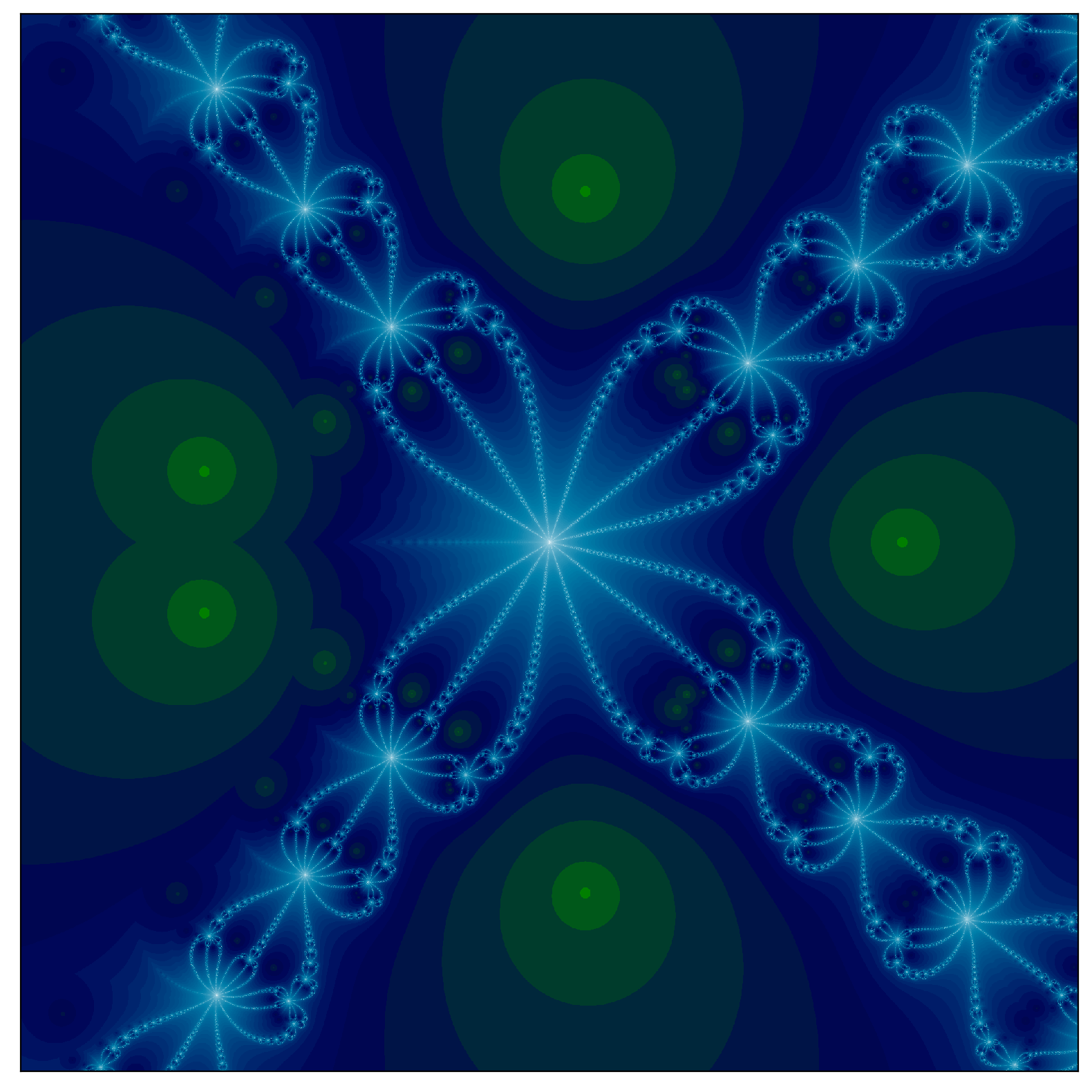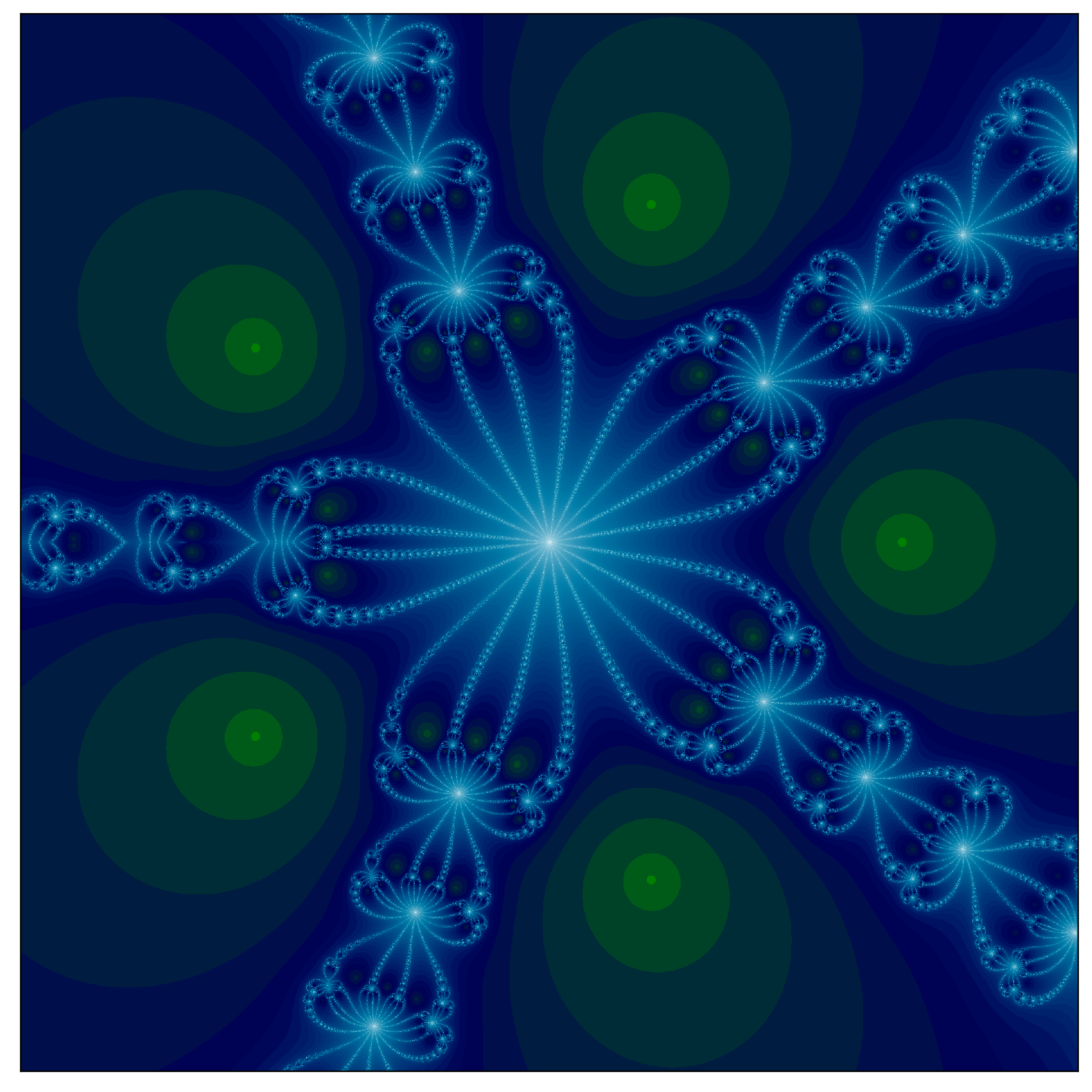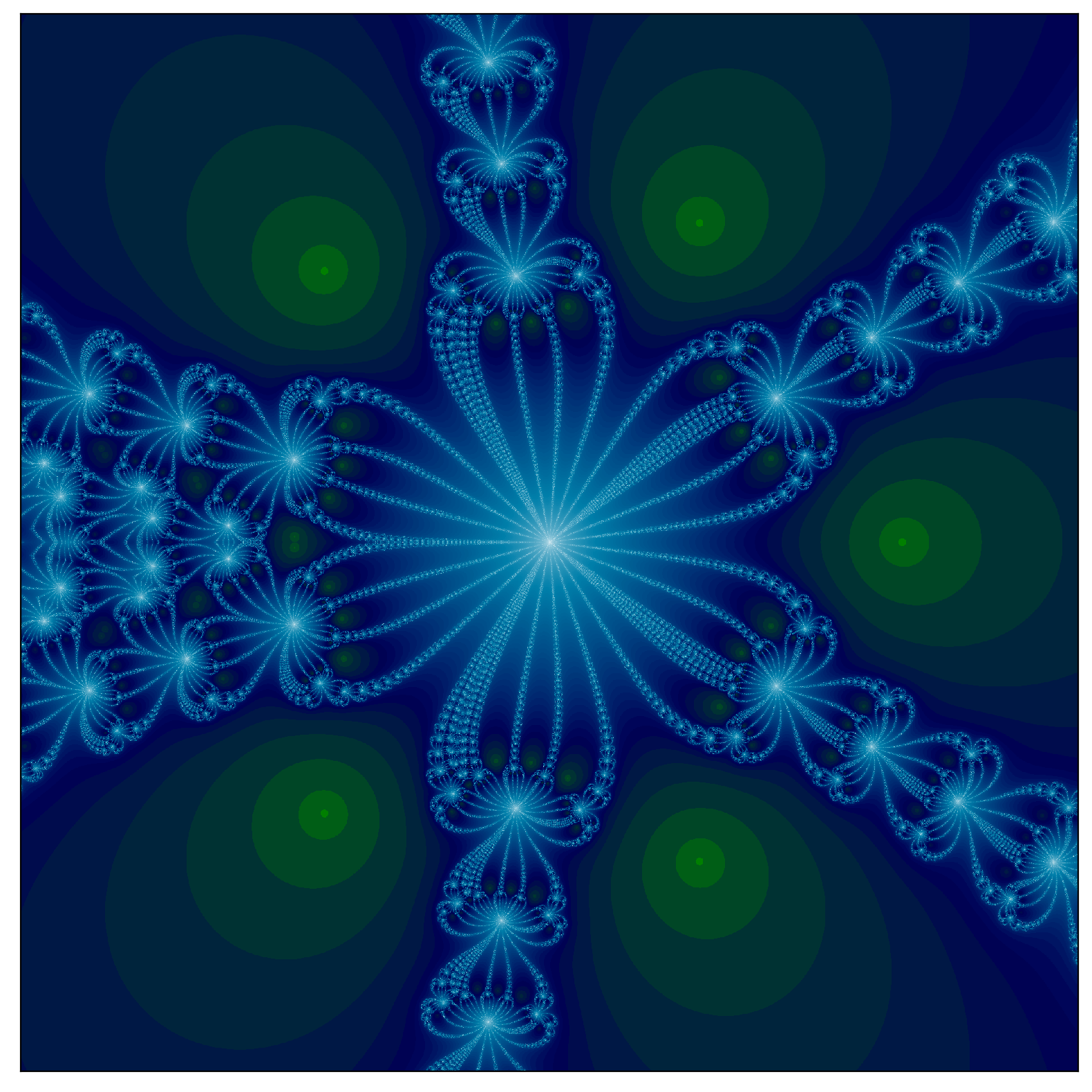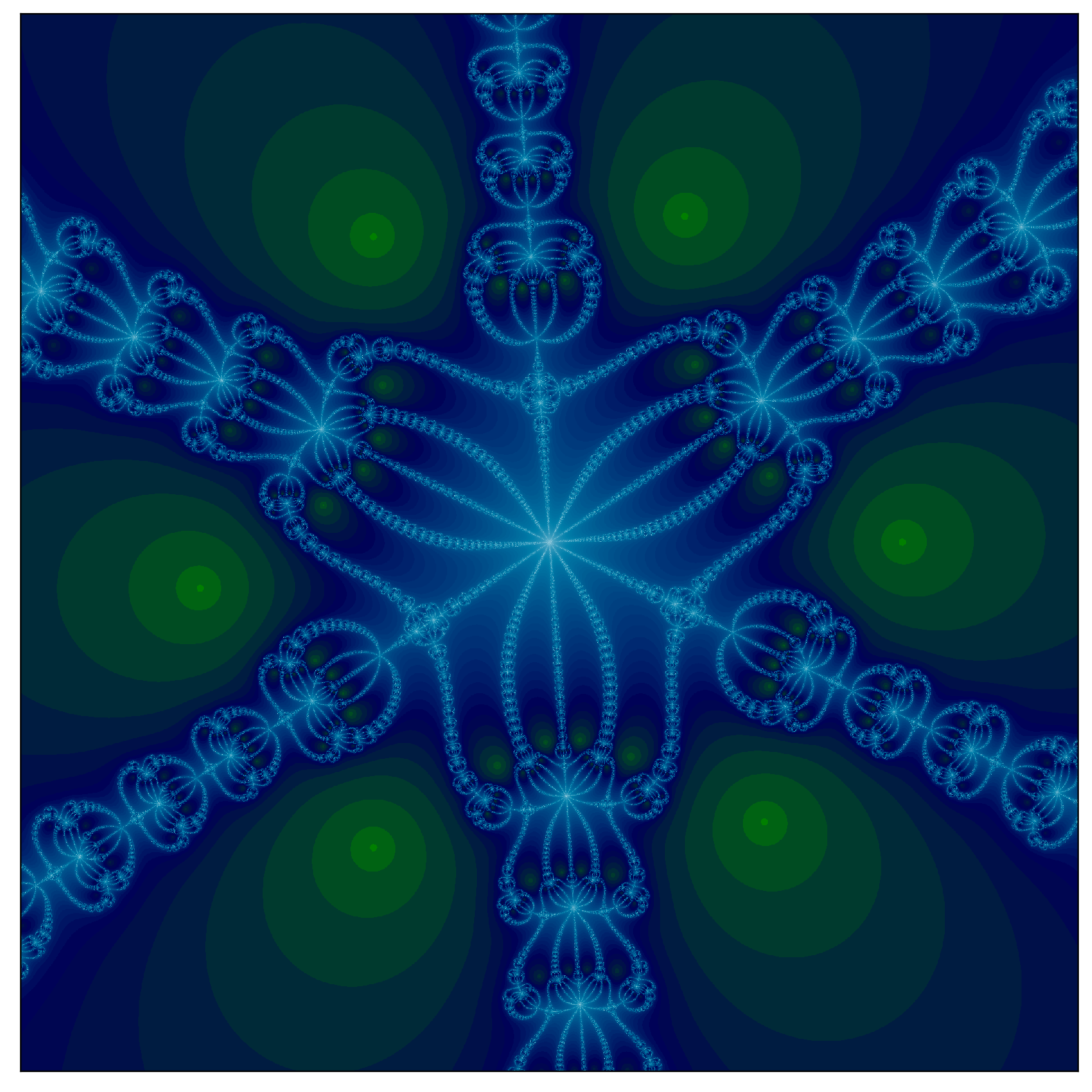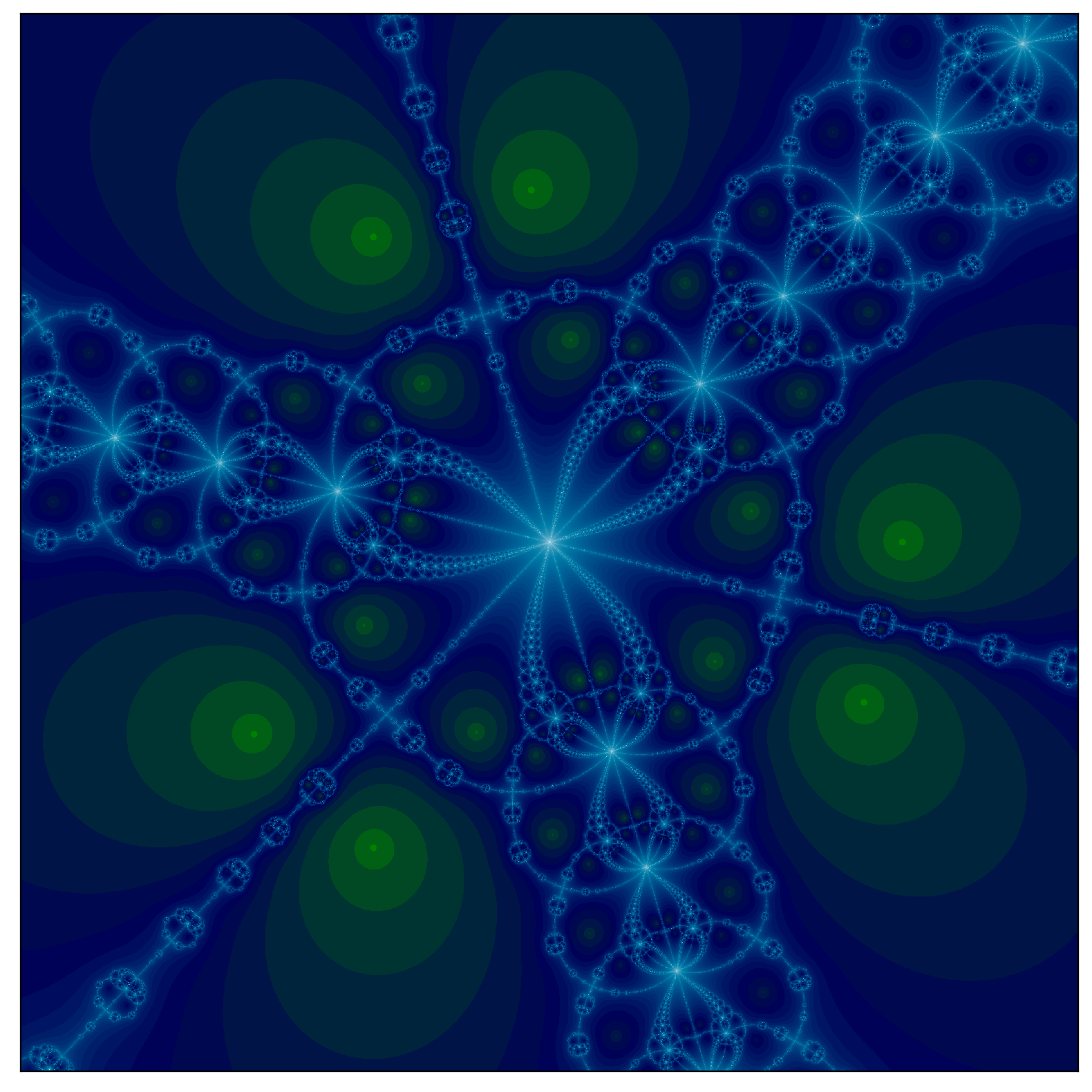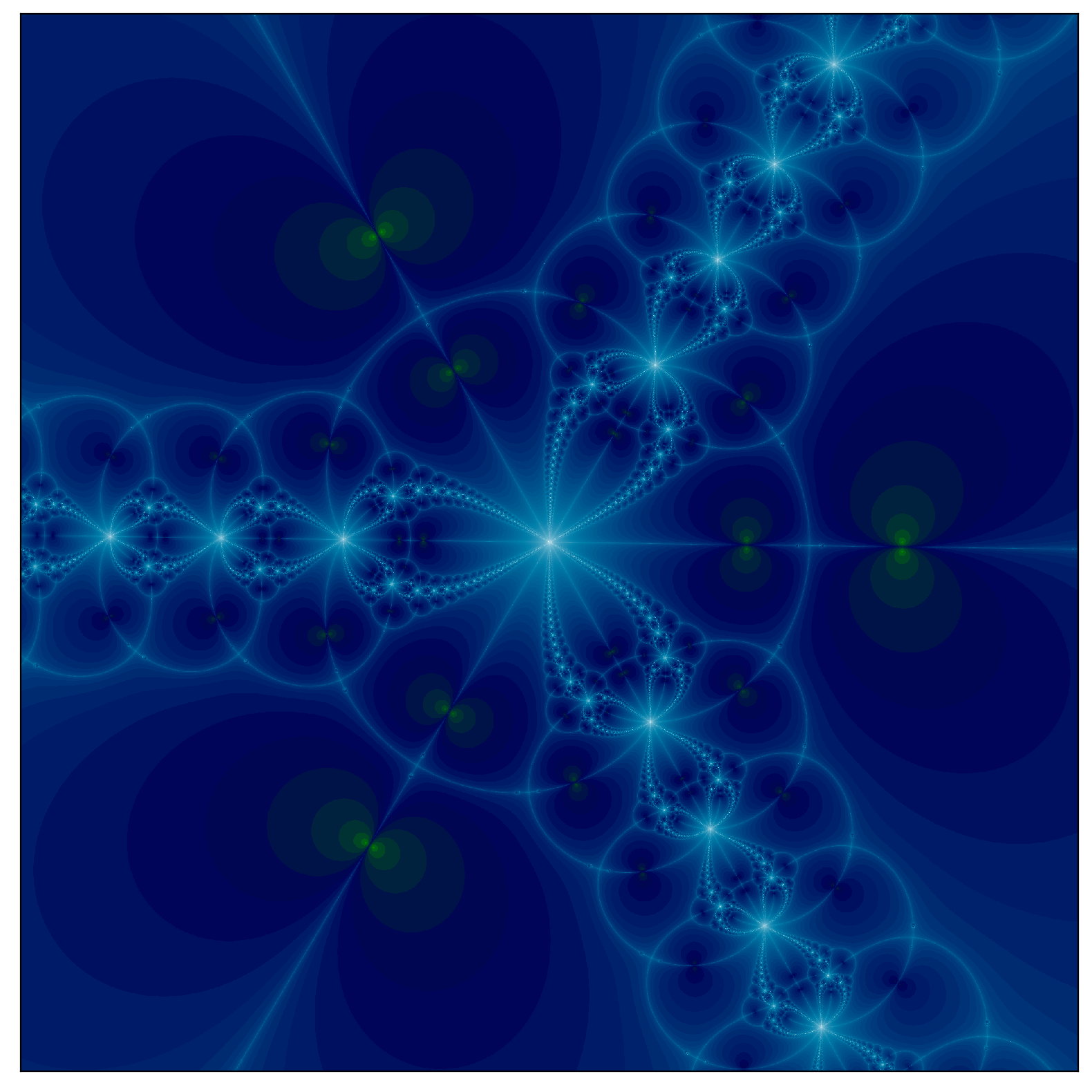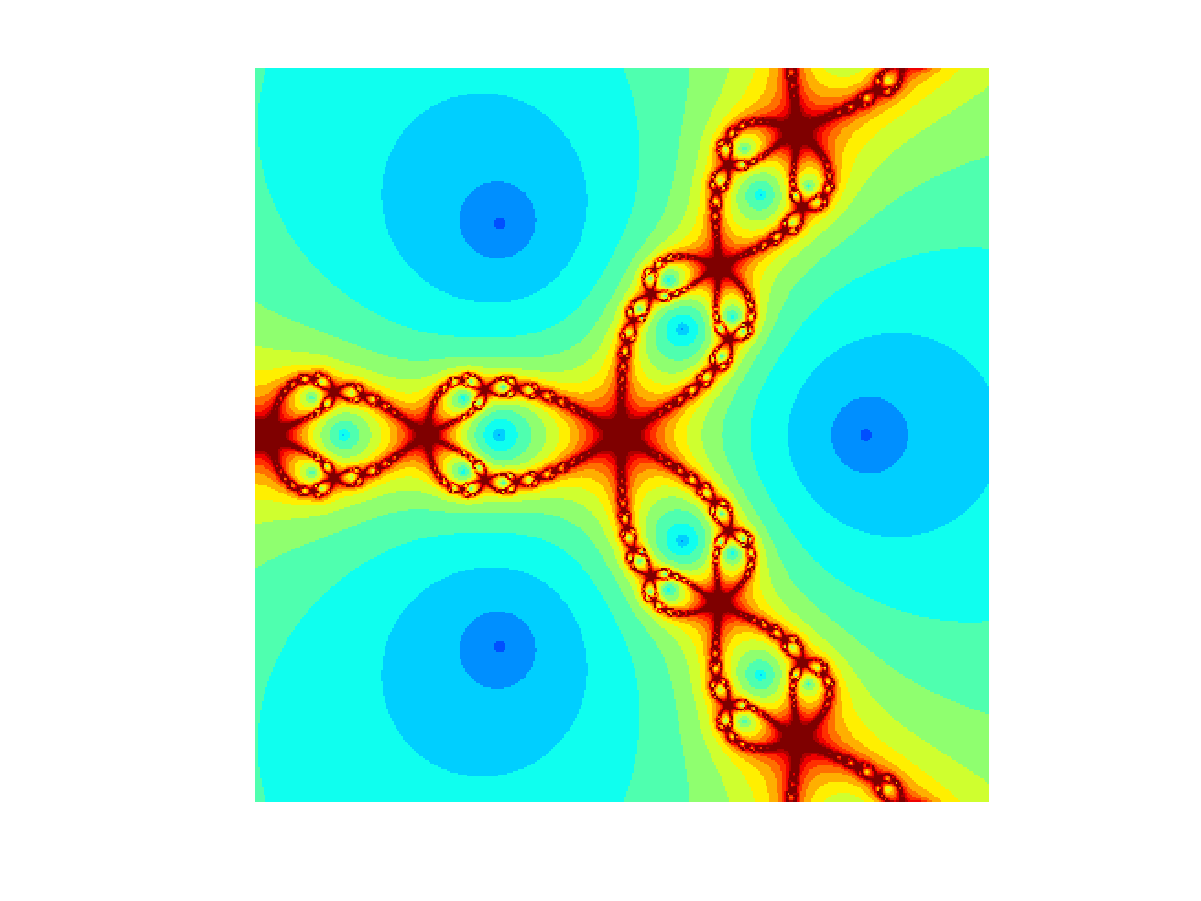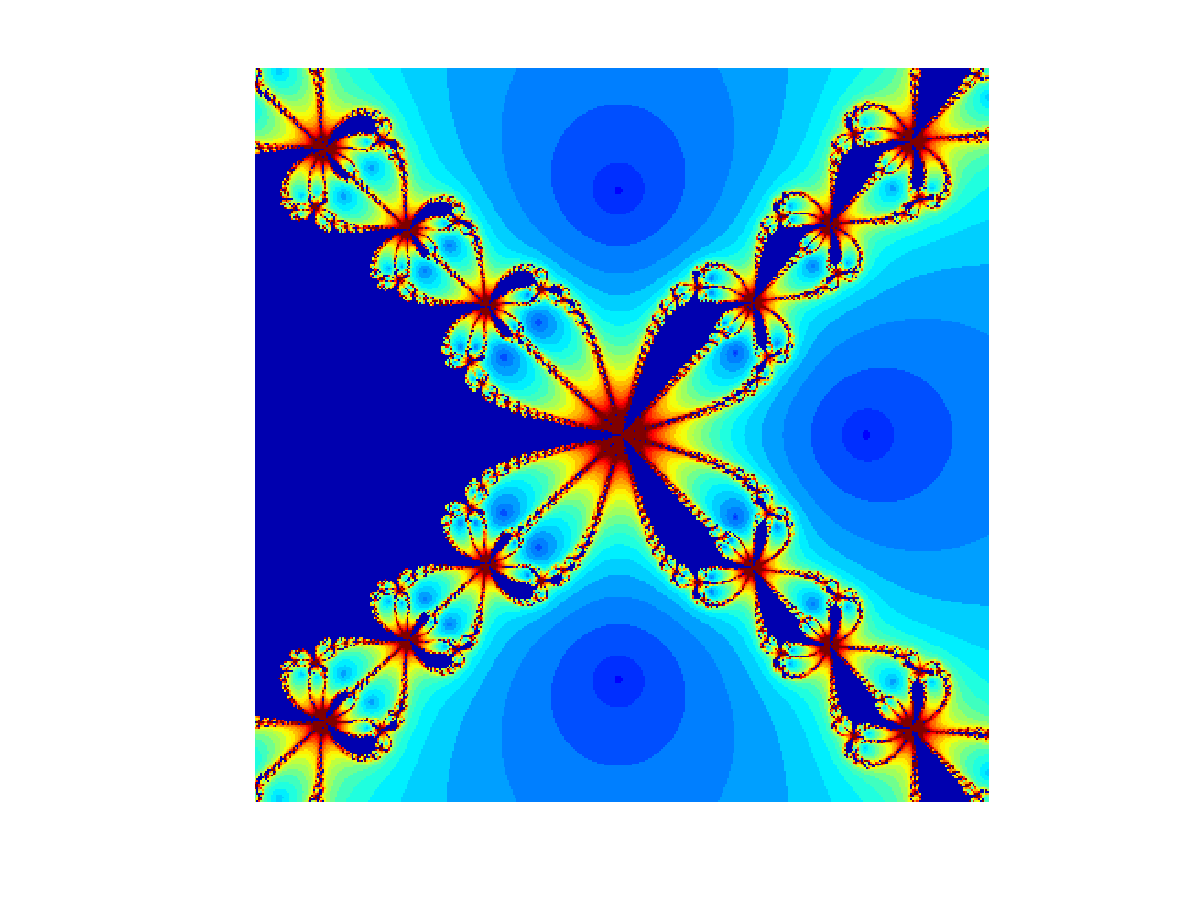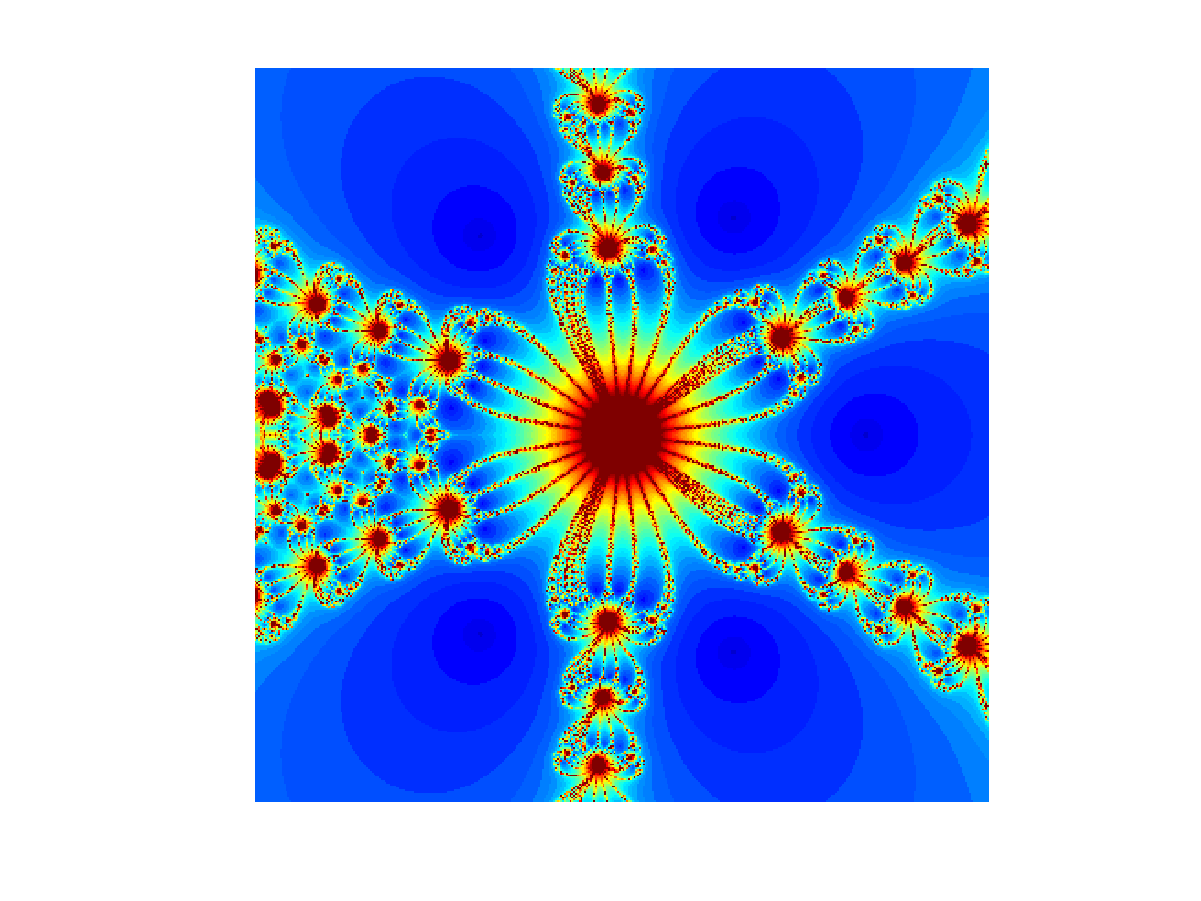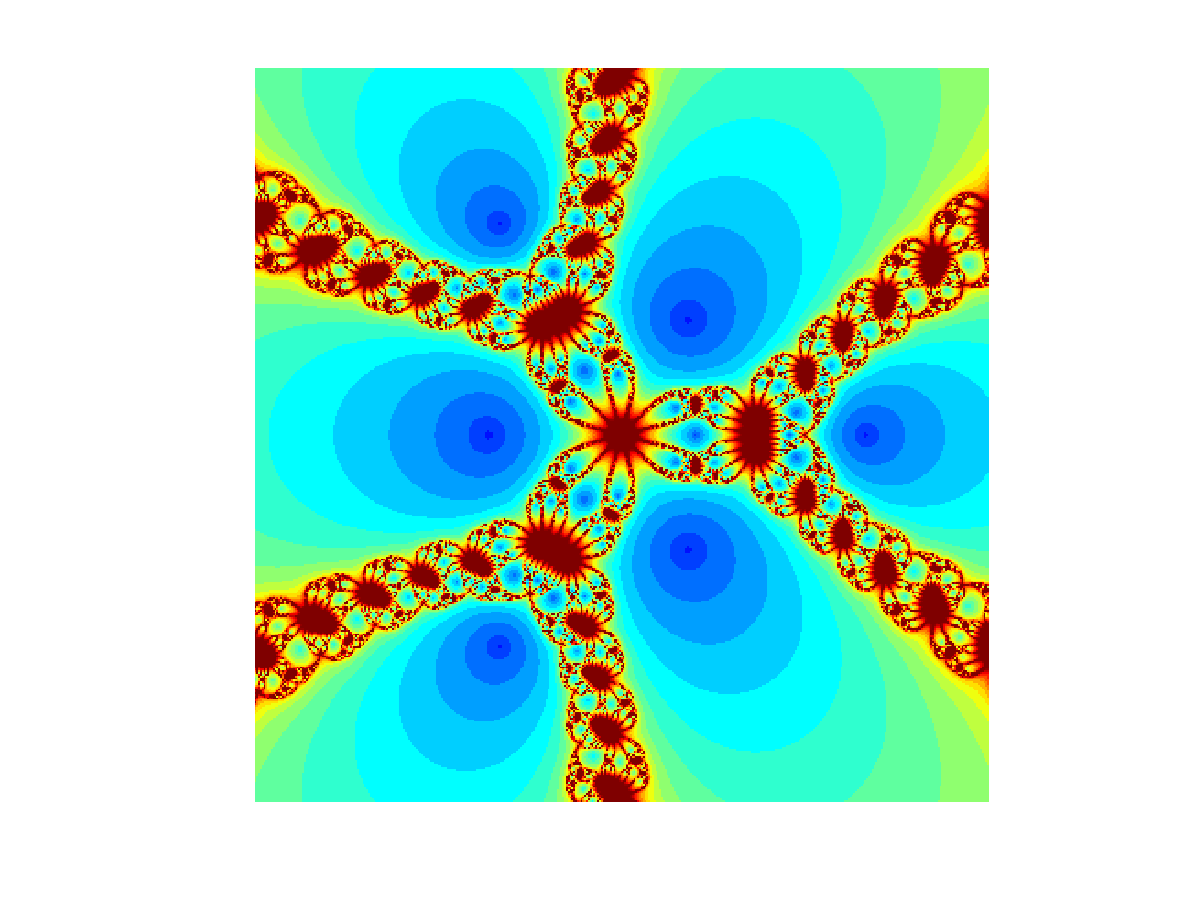Series of fractals obtained with Newton's method in the complex plane. Click on any image in the upper two rows to see it in 2K resolution. Here are three animations: One, two, and three.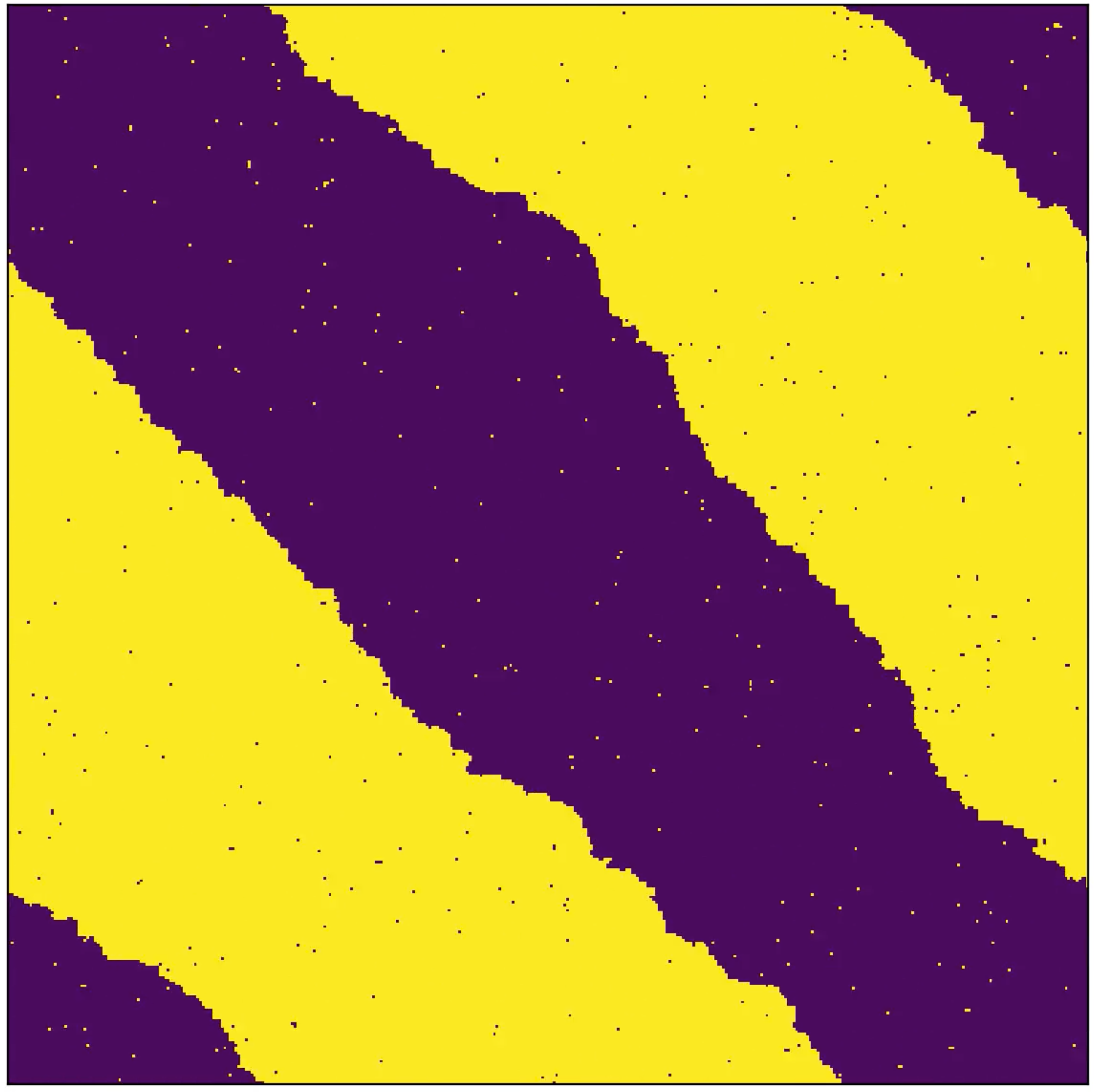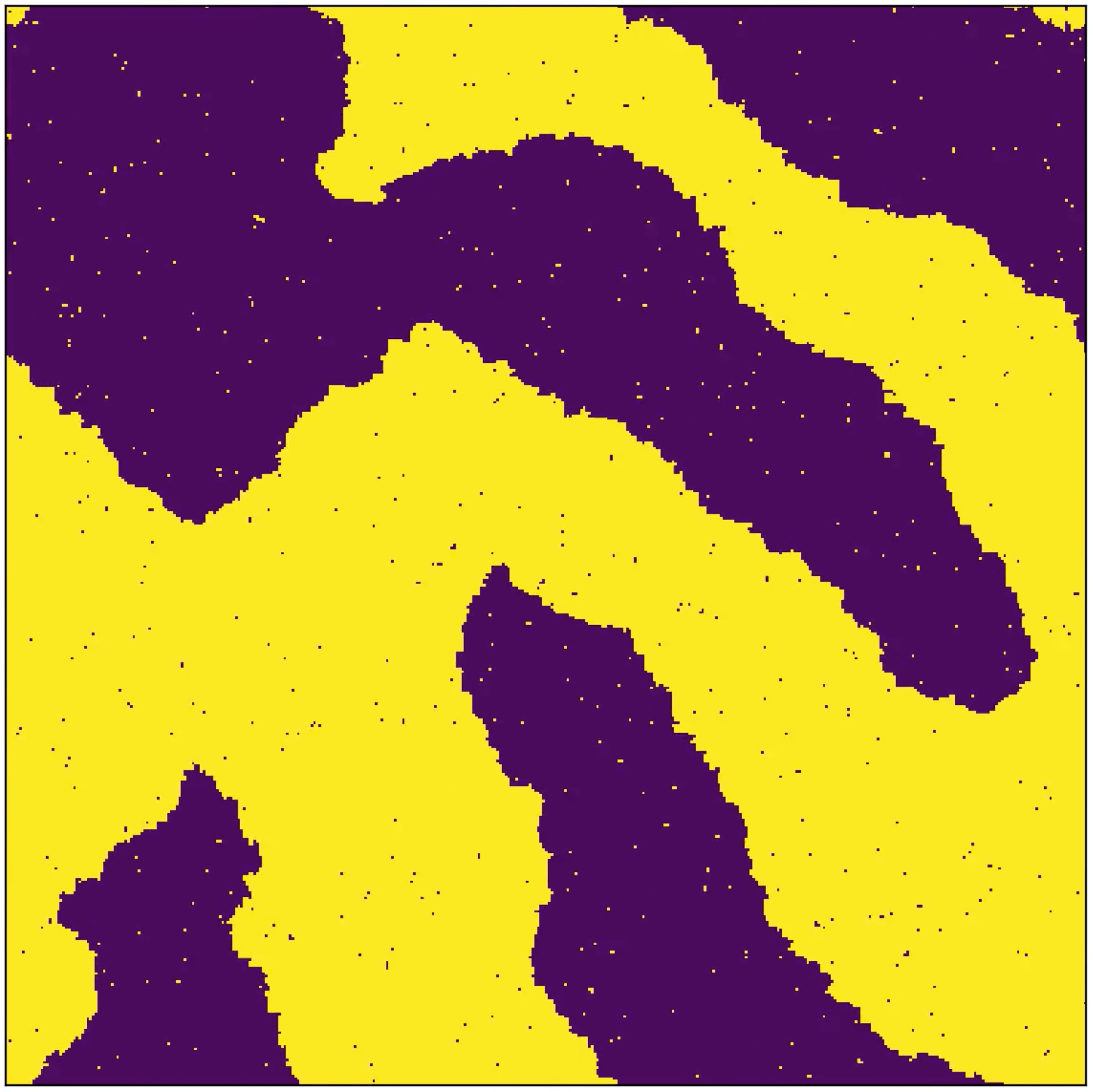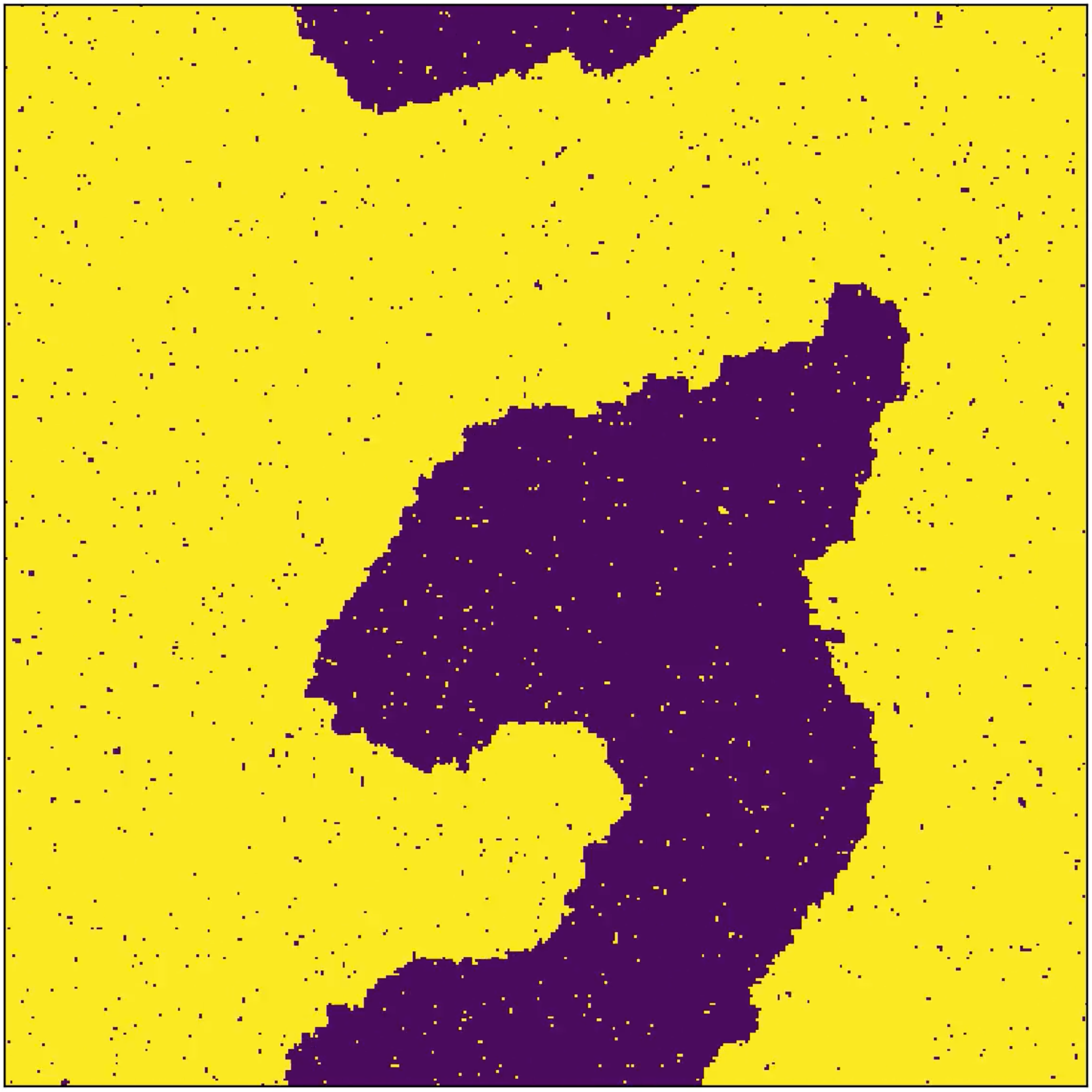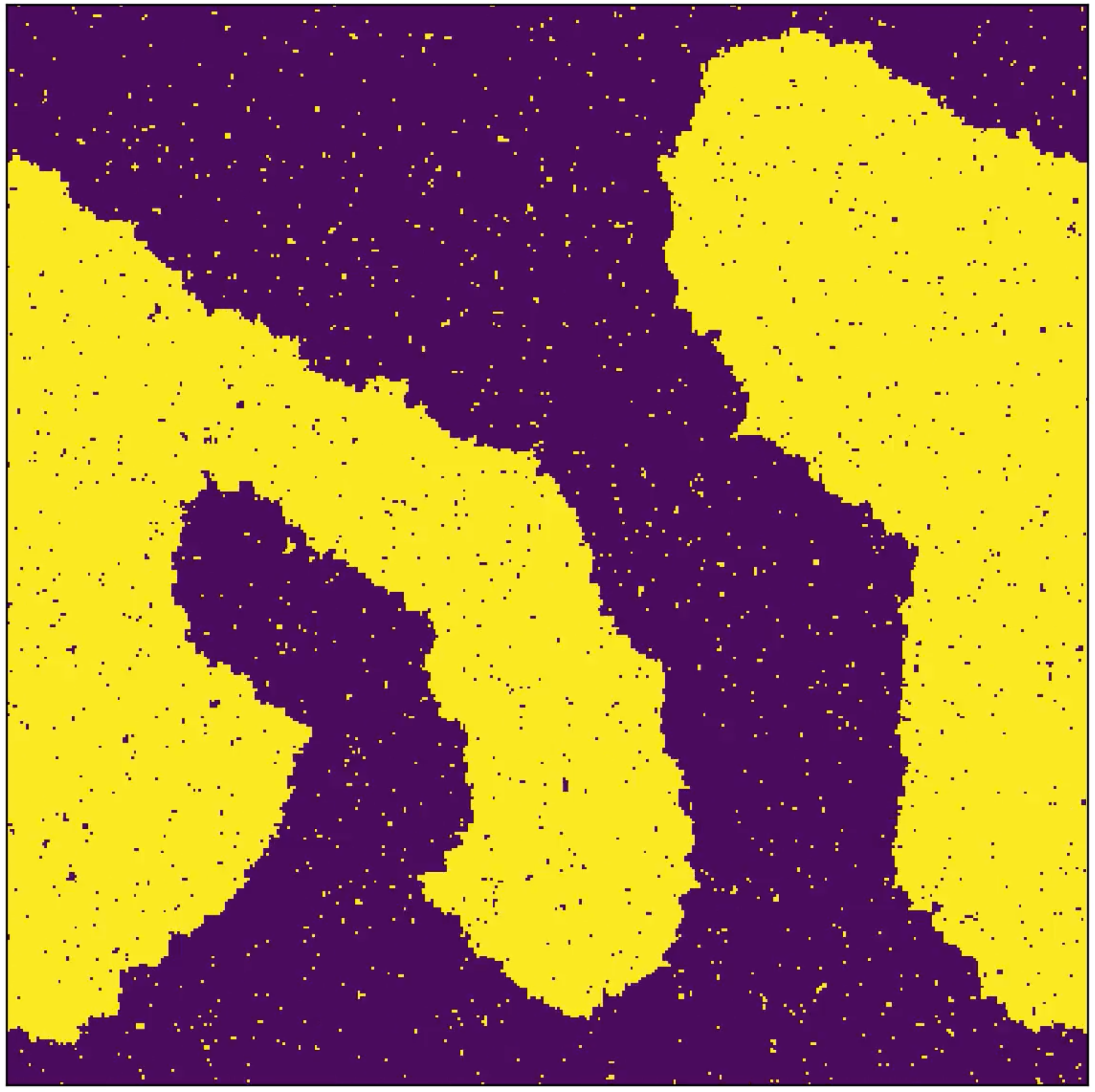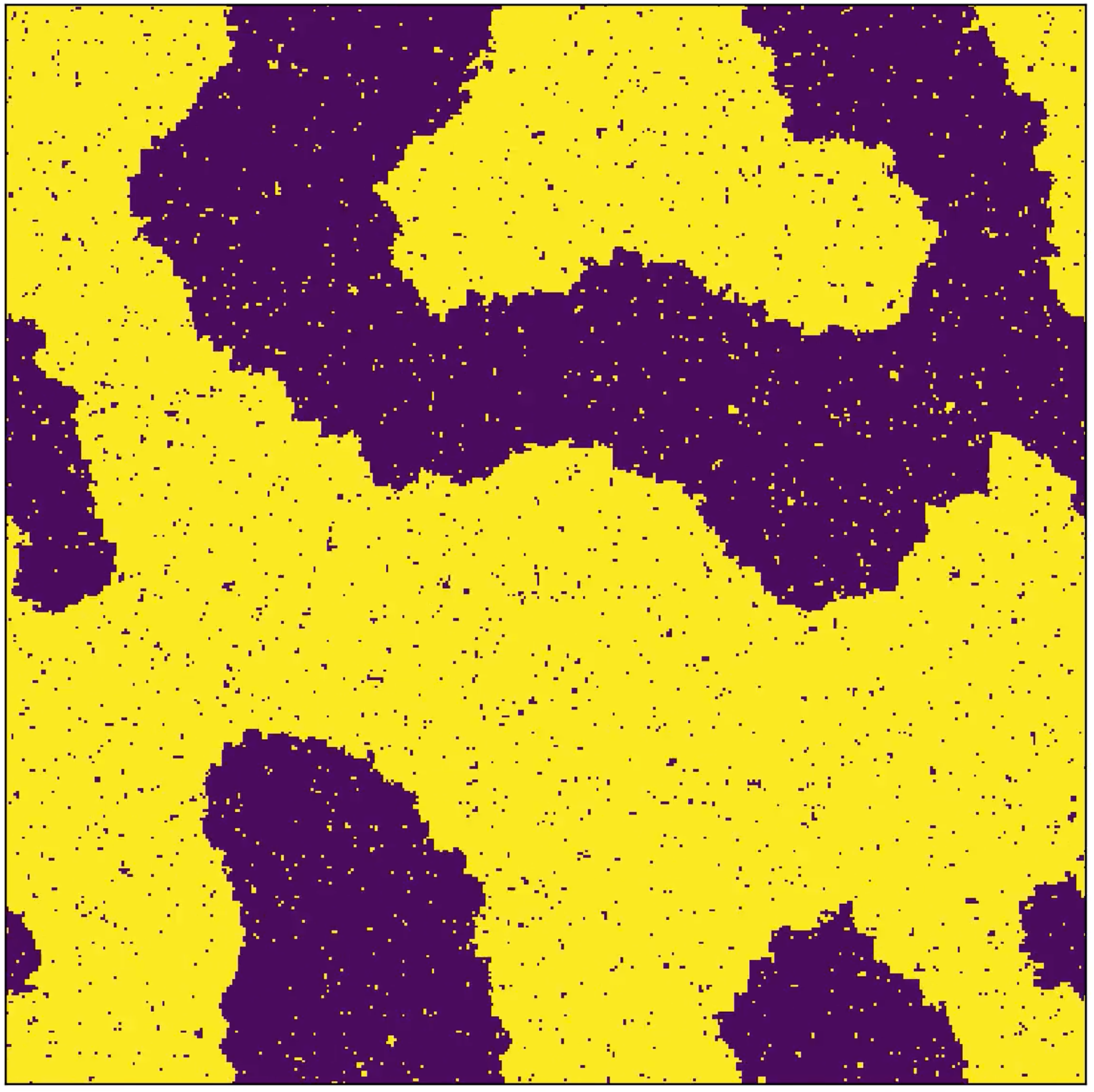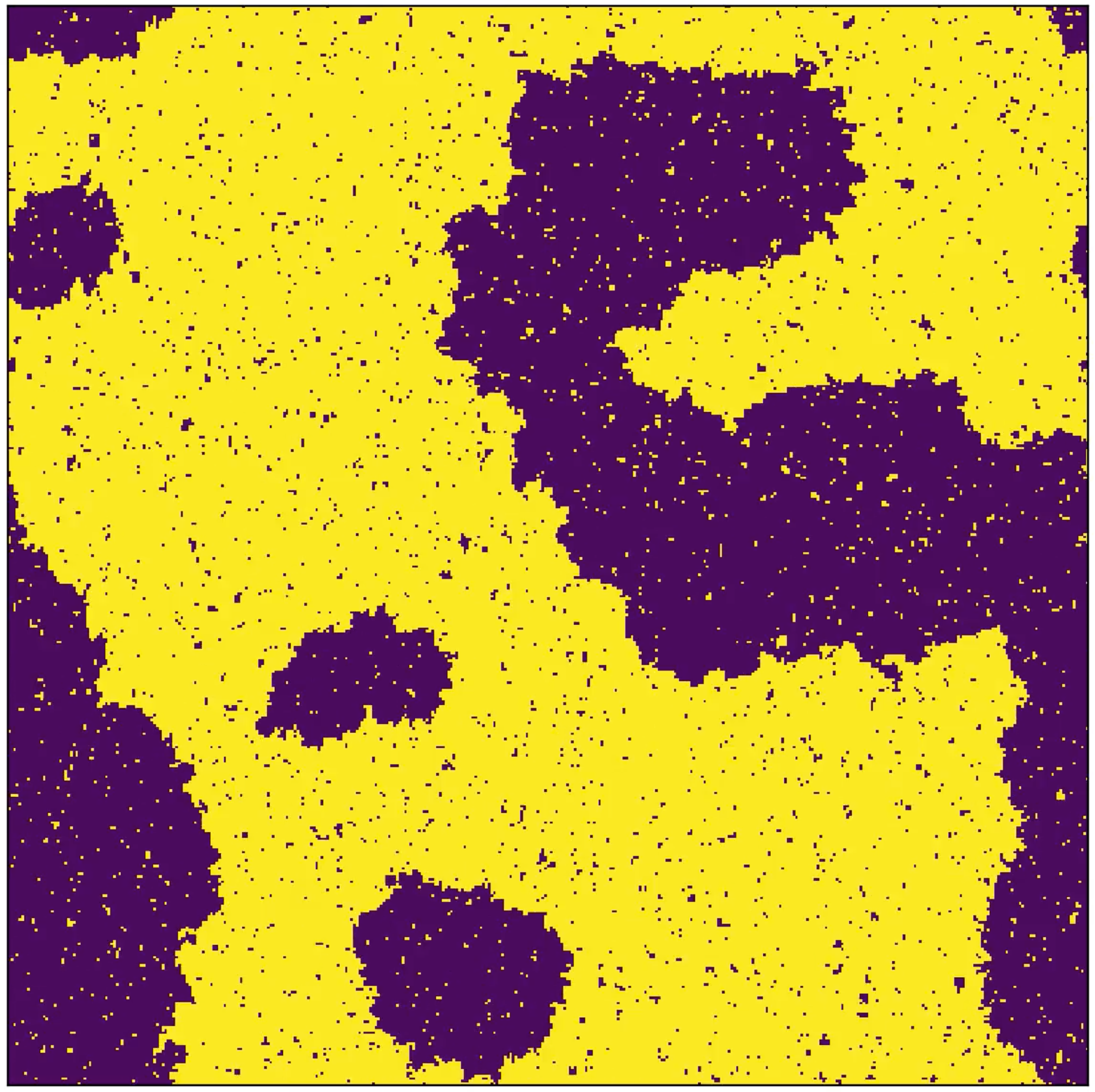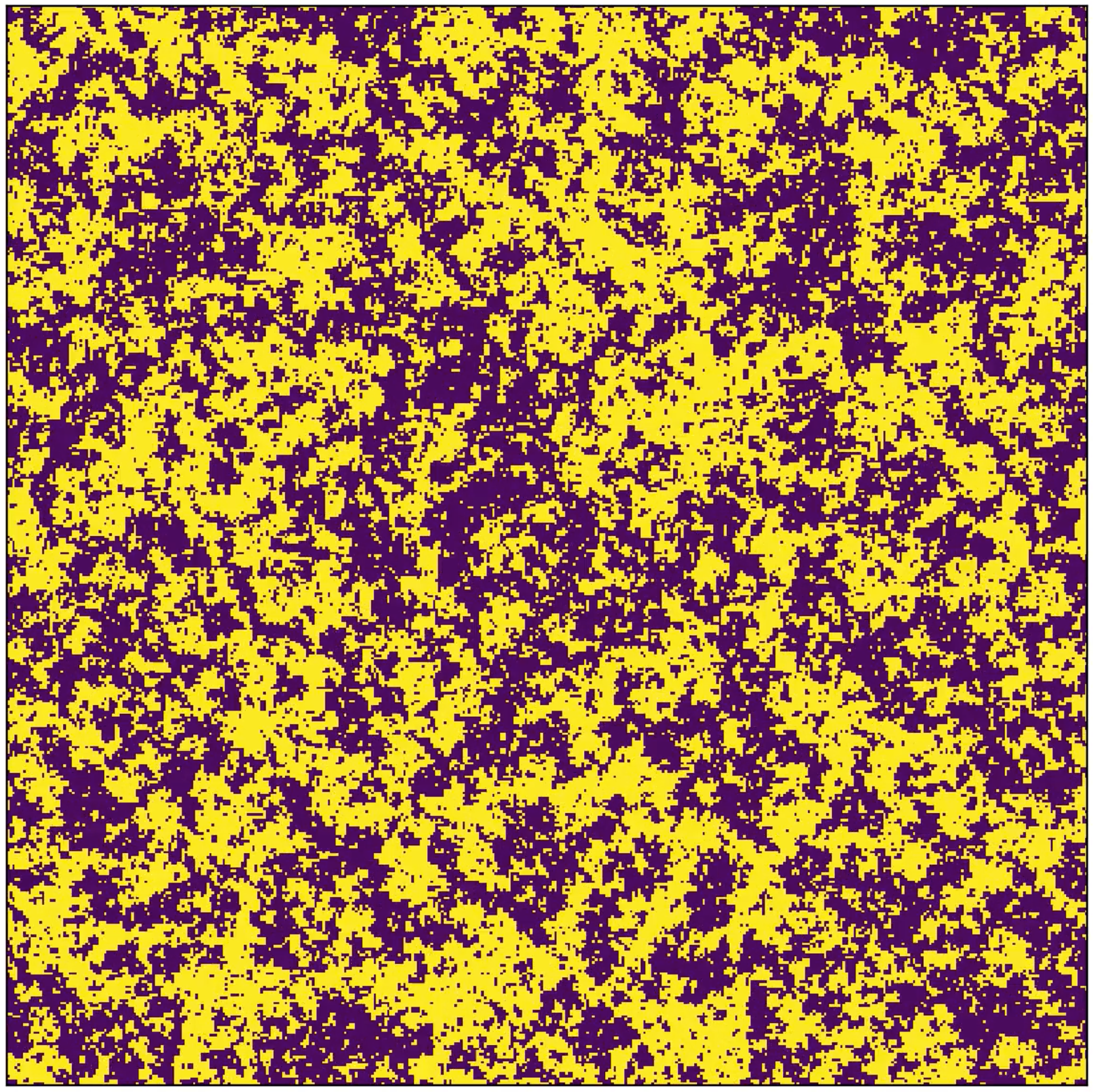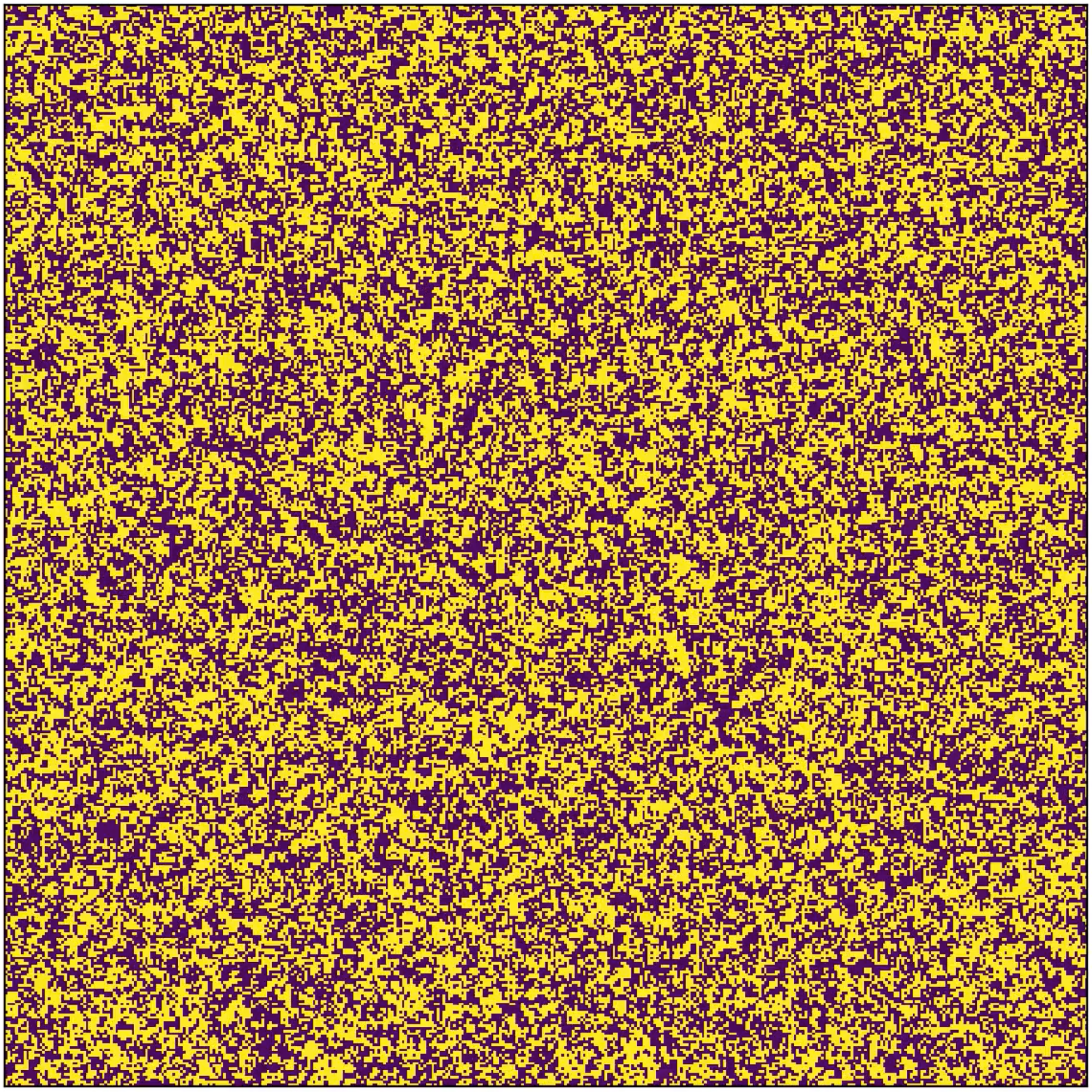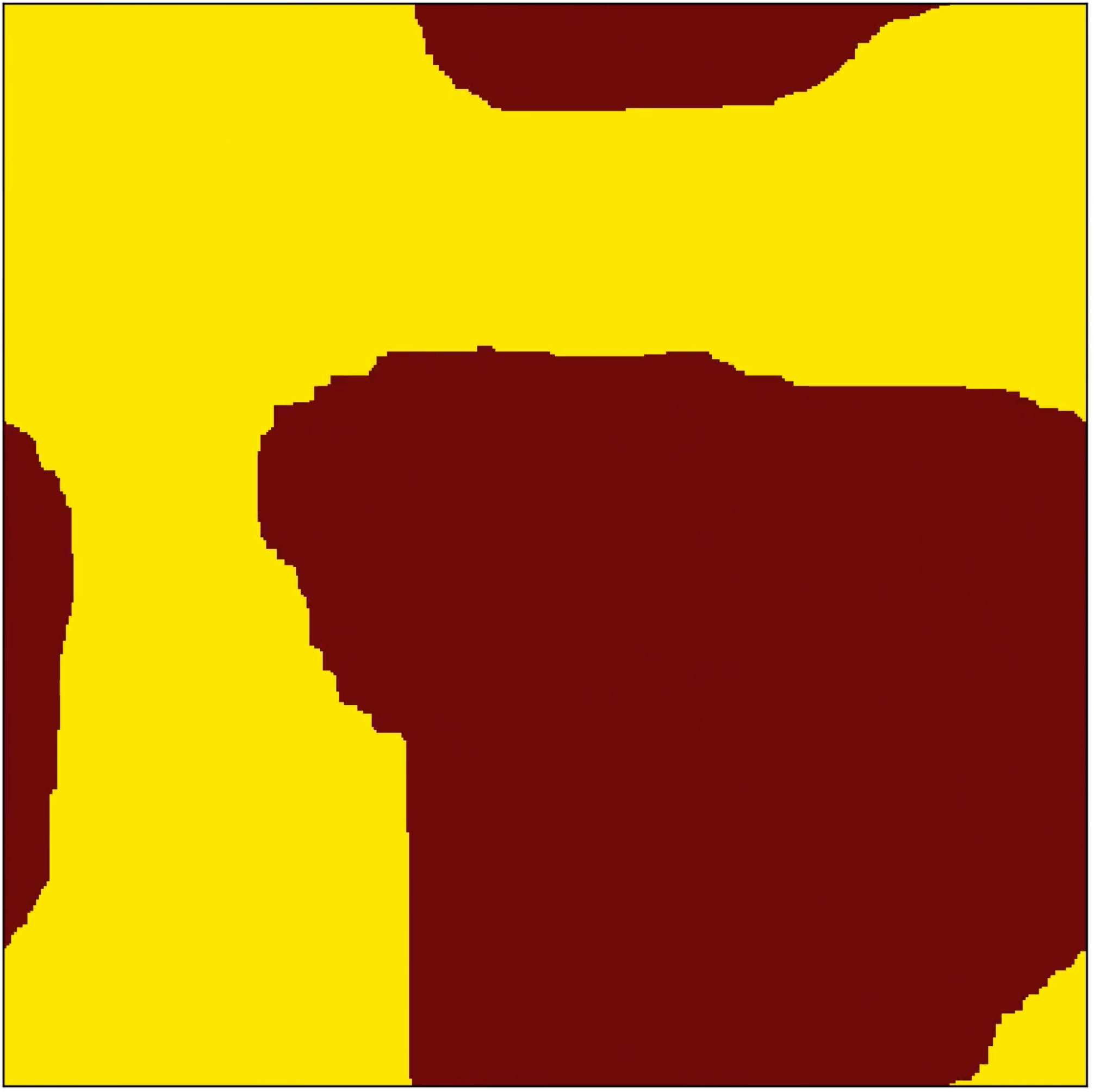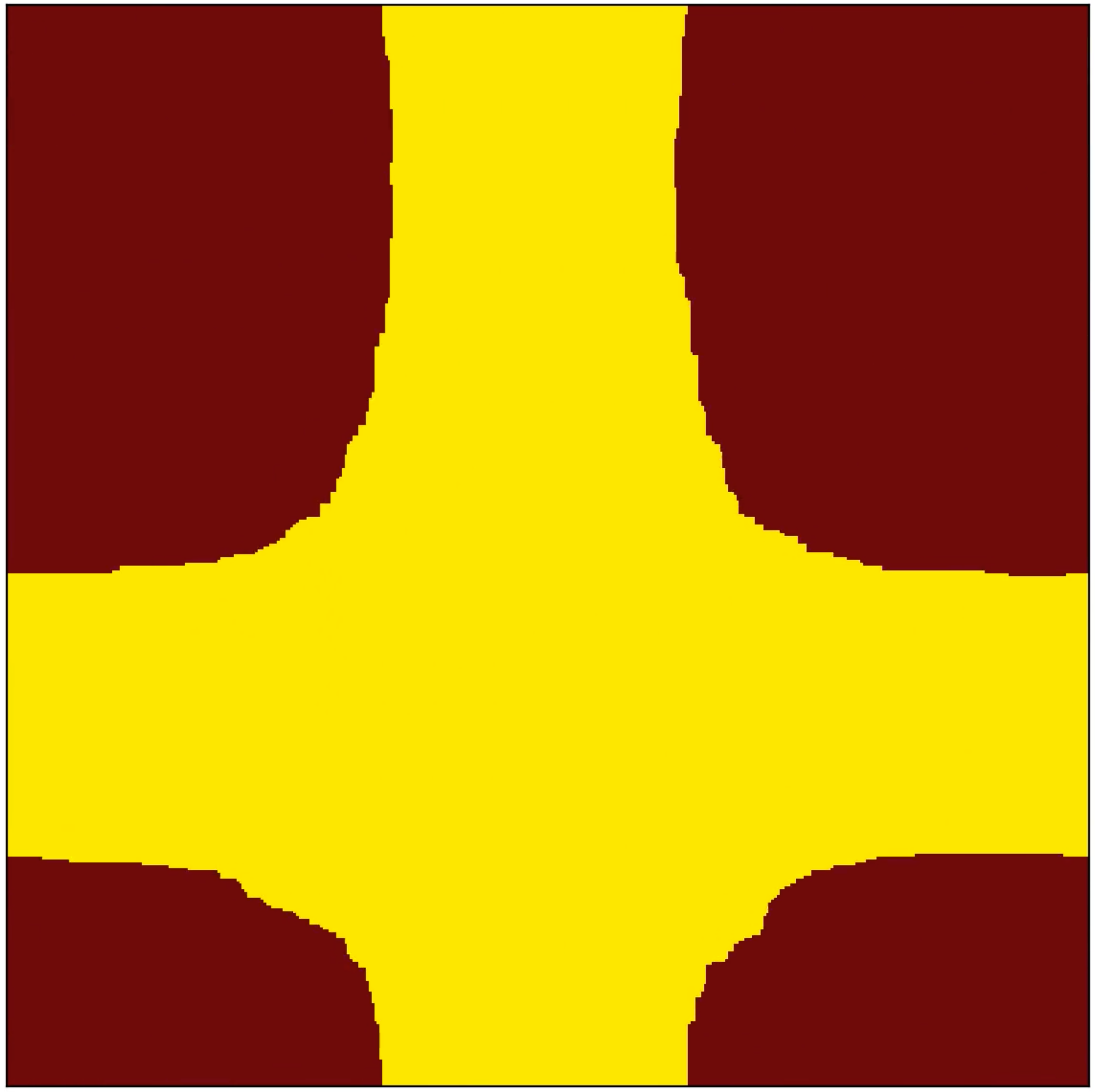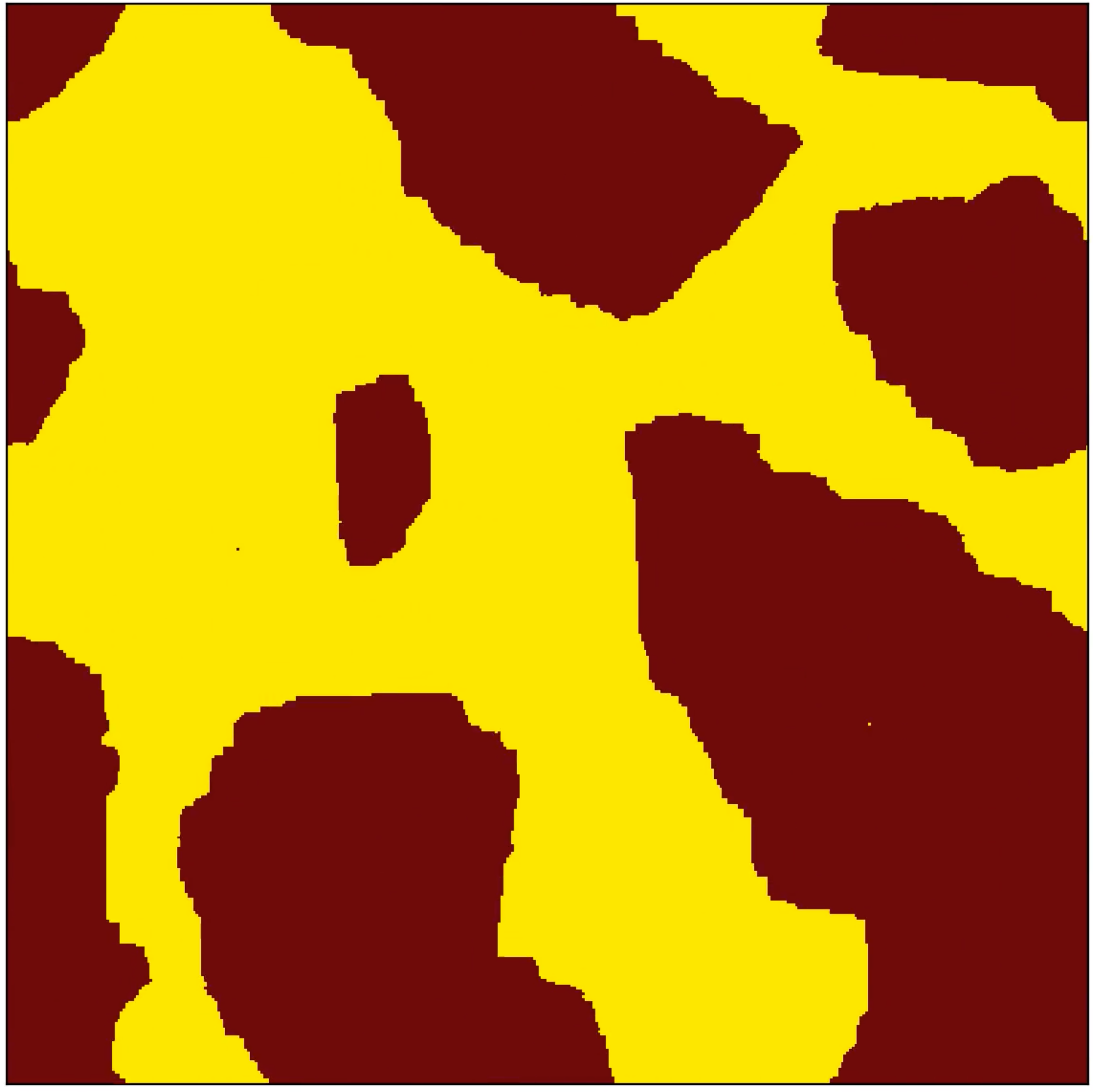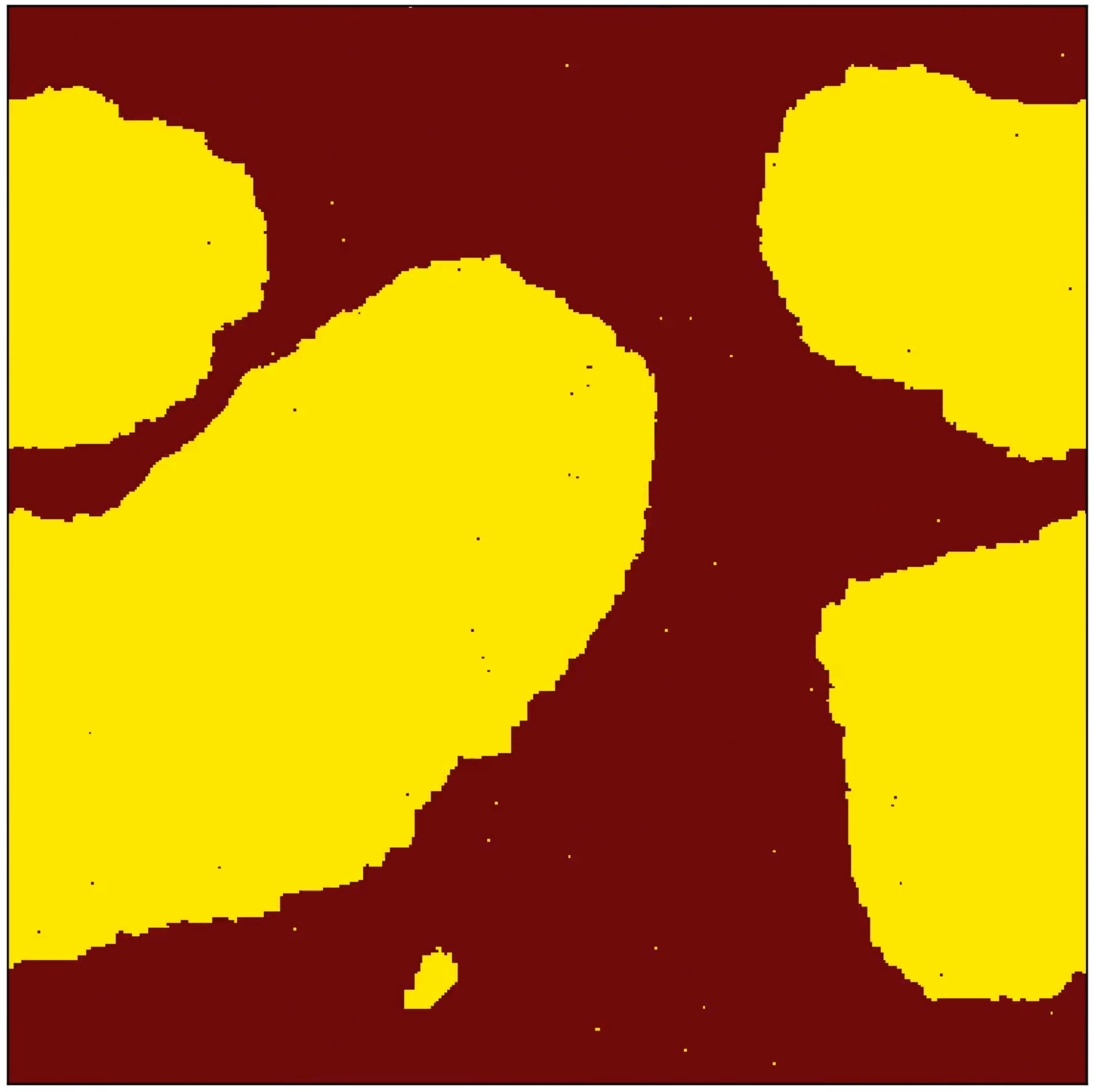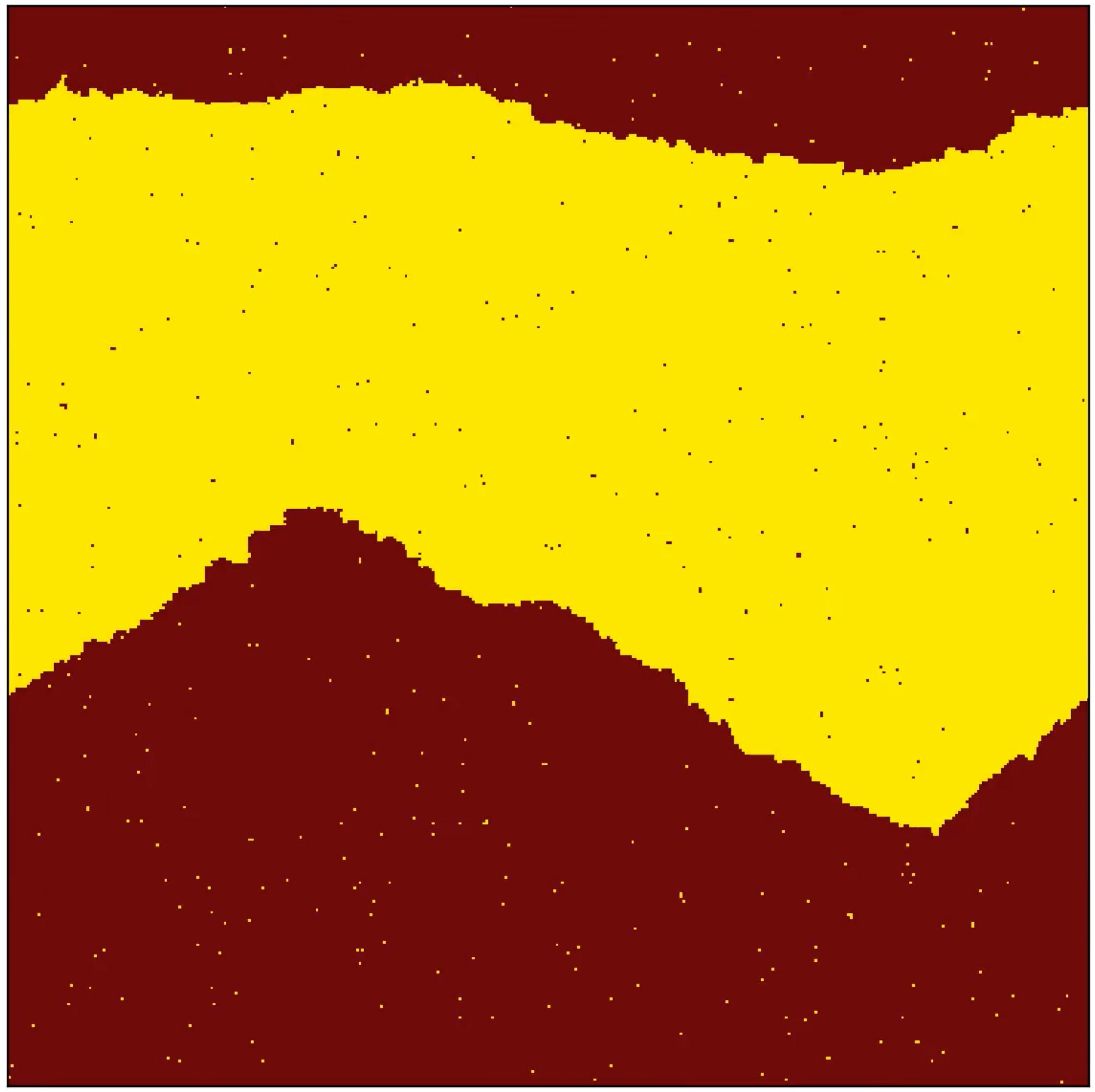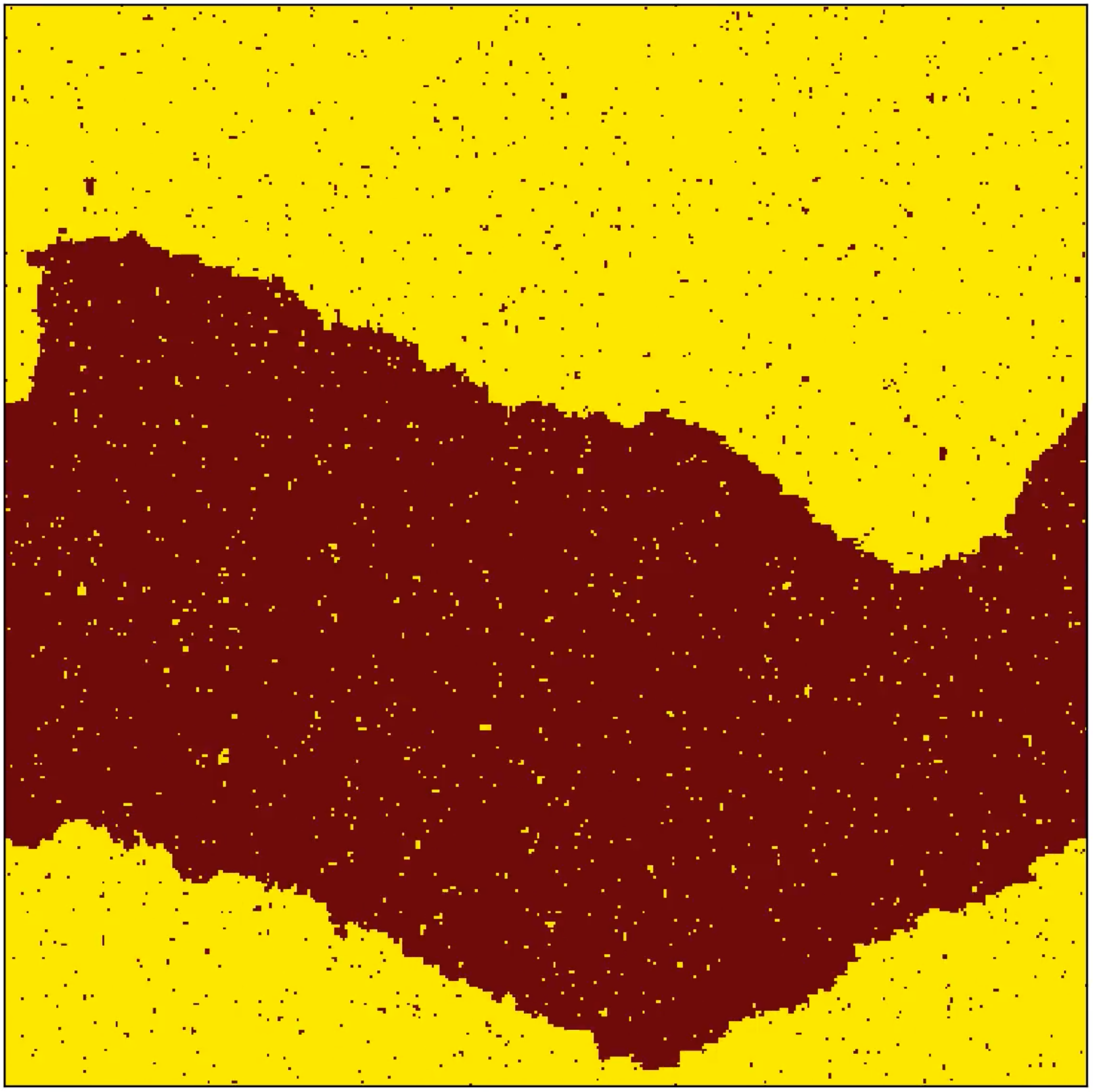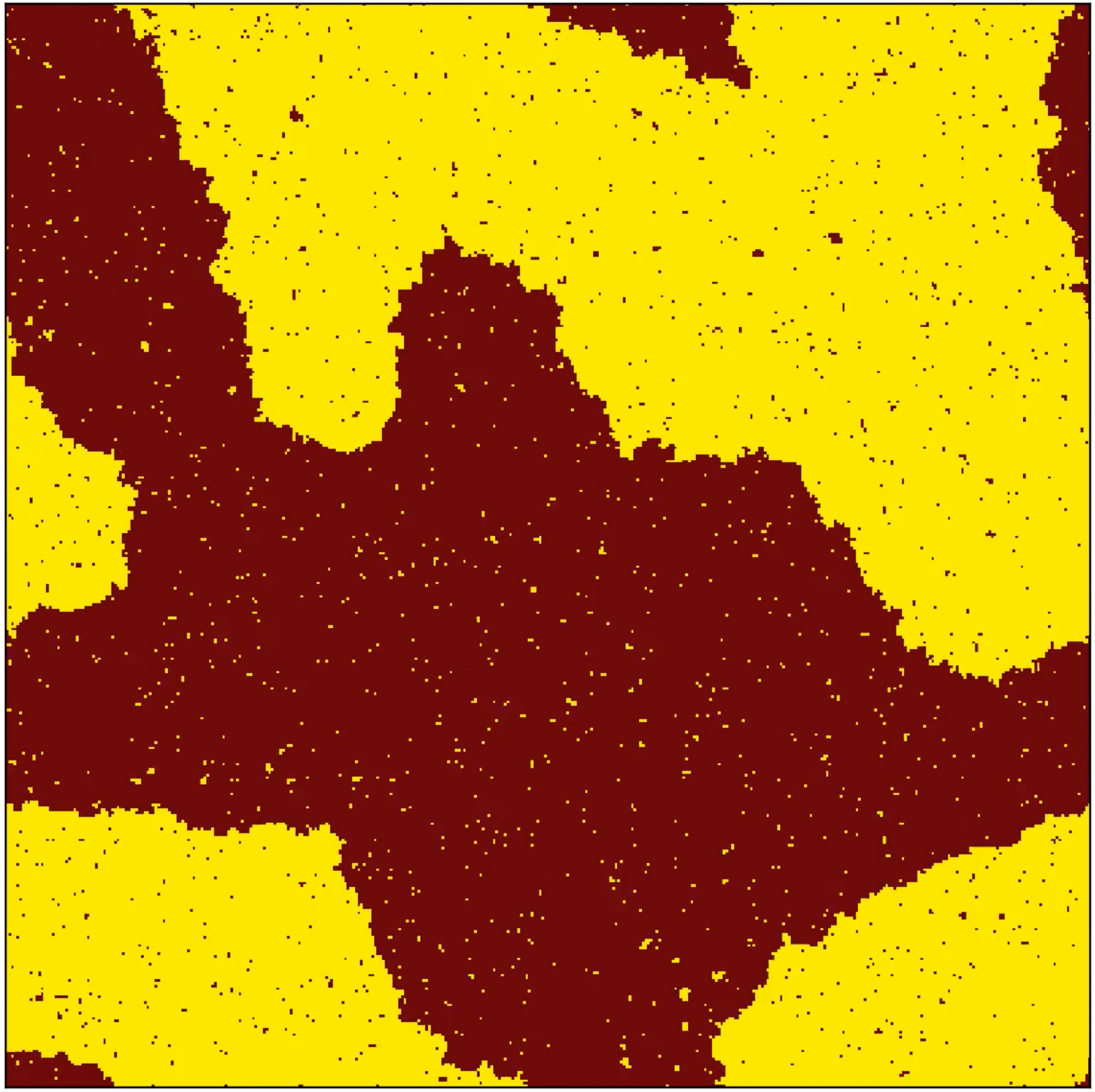Monte Carlo simulations of a 2D Ising spin model. In both rows of images, the temperature increases from left to right. The images in the lower row were generated by constraining the magnetization to be 50%. Click on every image to start the corresponding animation. Here is the Jupyter notebook that was used to make these animations.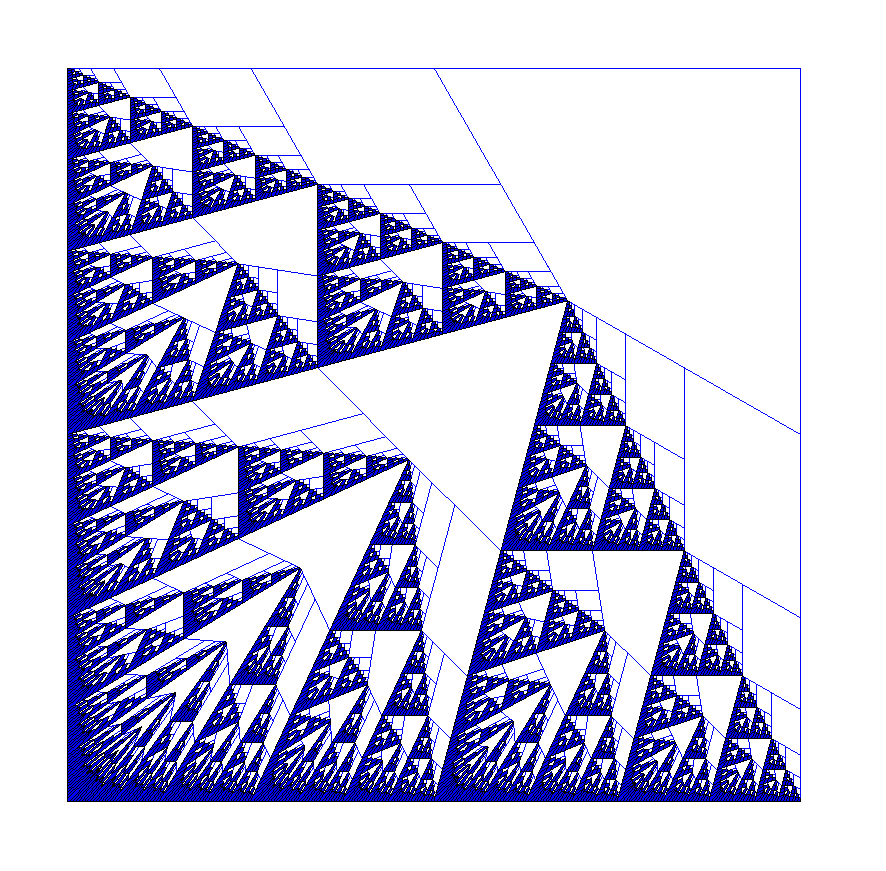Triangular fractal (click on image to start movie)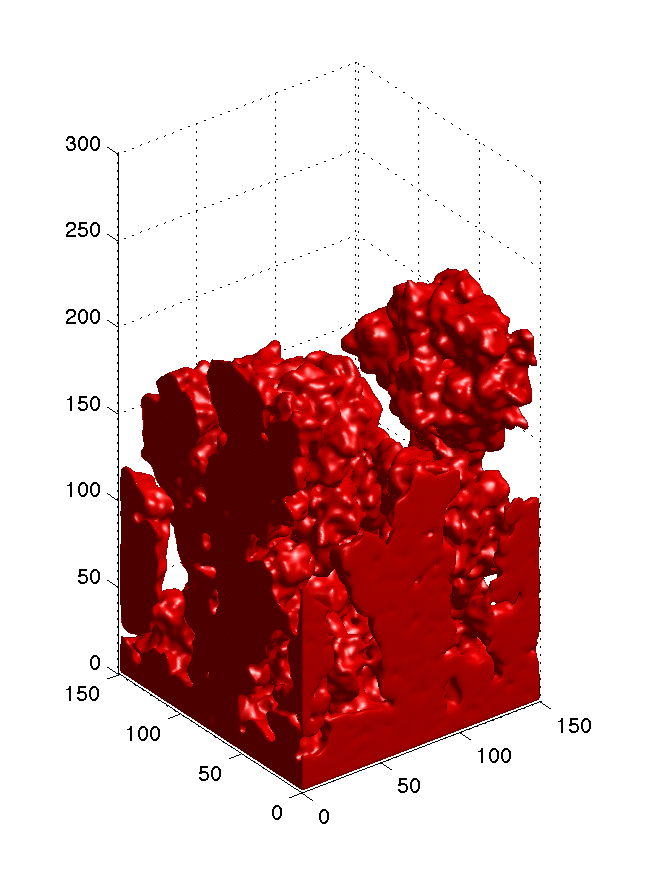Aggregation in 3D (click on image to start movie)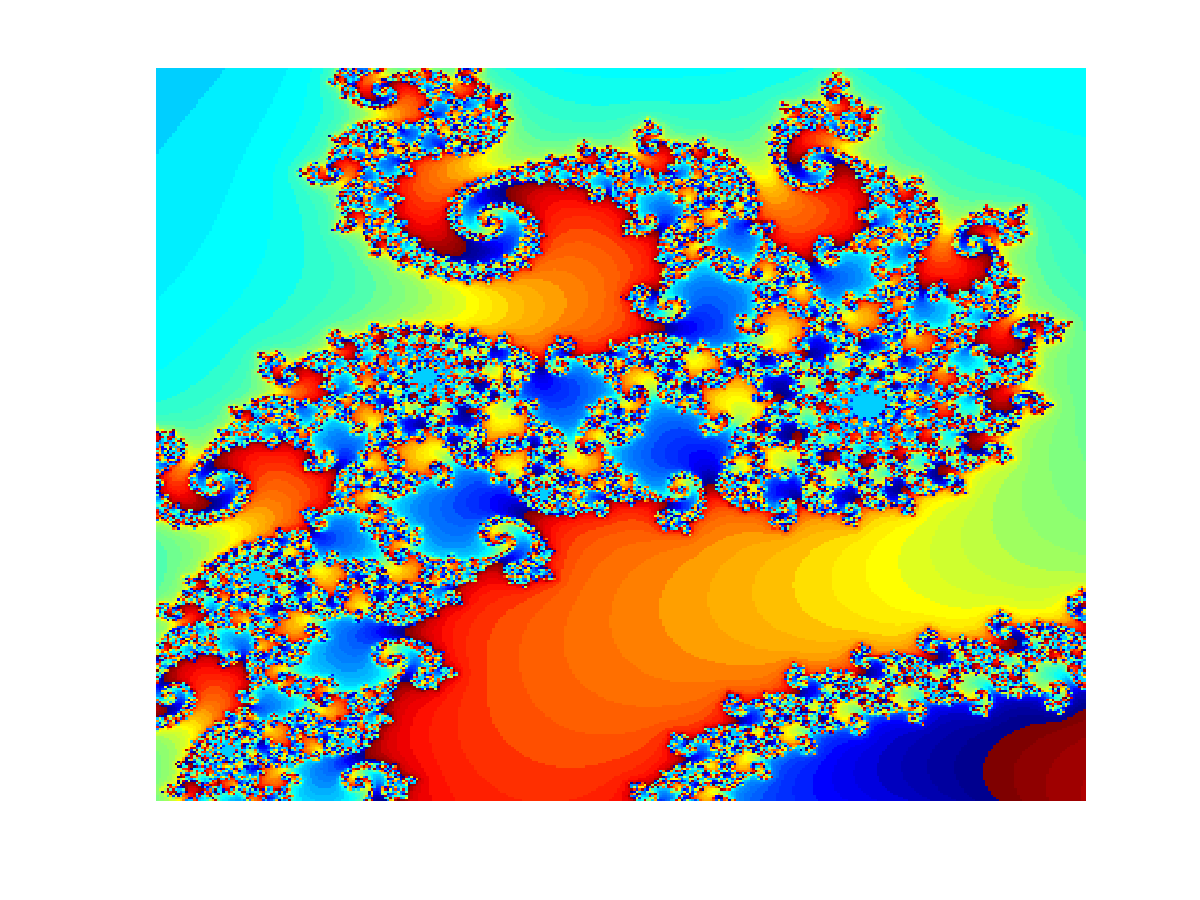Mandelbrot fractal (click on image to start movie)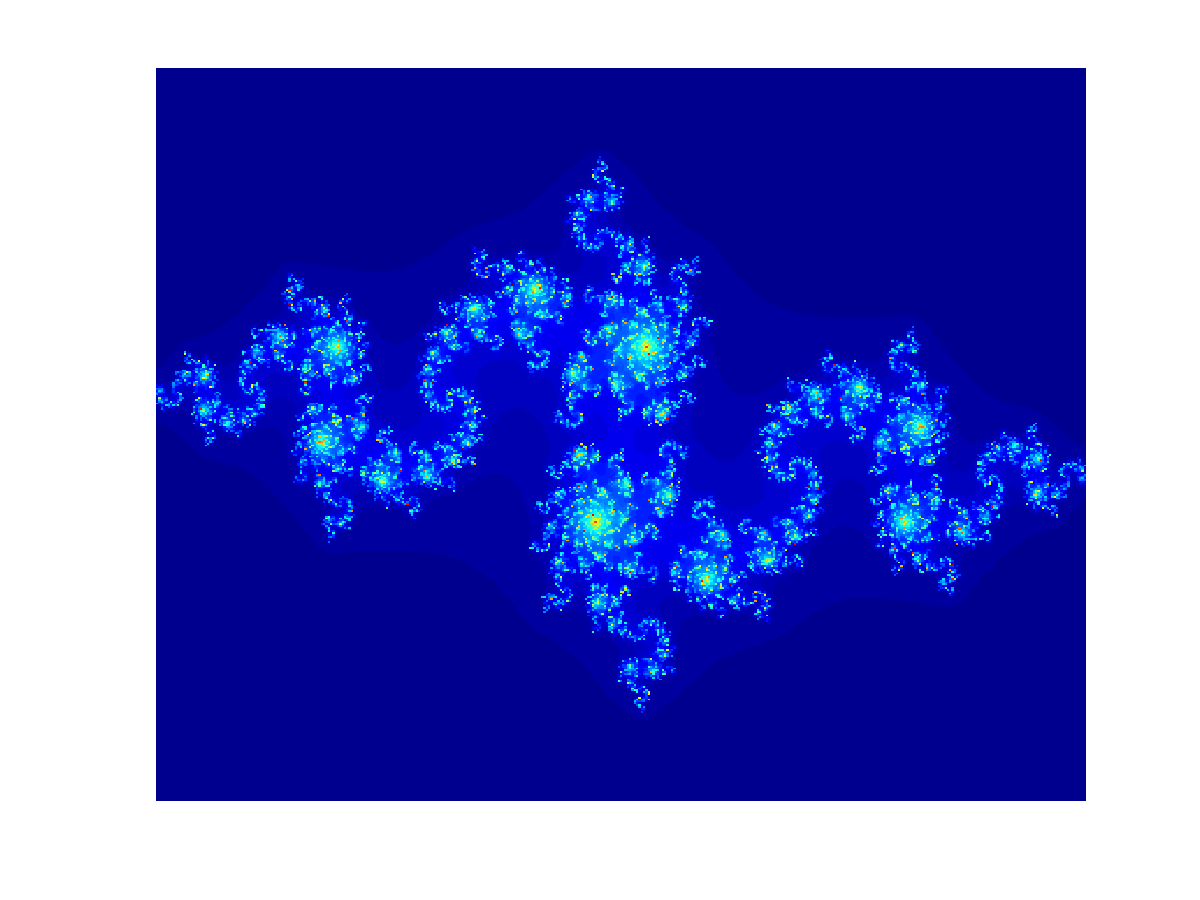Julia set (click on image to start movie)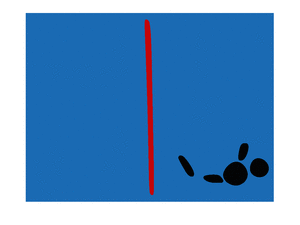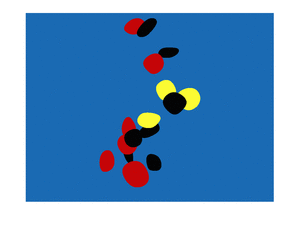In the foot steps of Joan Miro (Higher resolution available: left and right)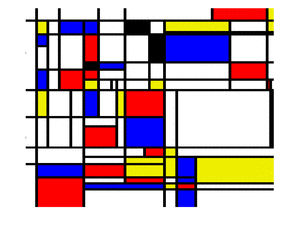In the spirit of Piet Mondrian (higher resolution available)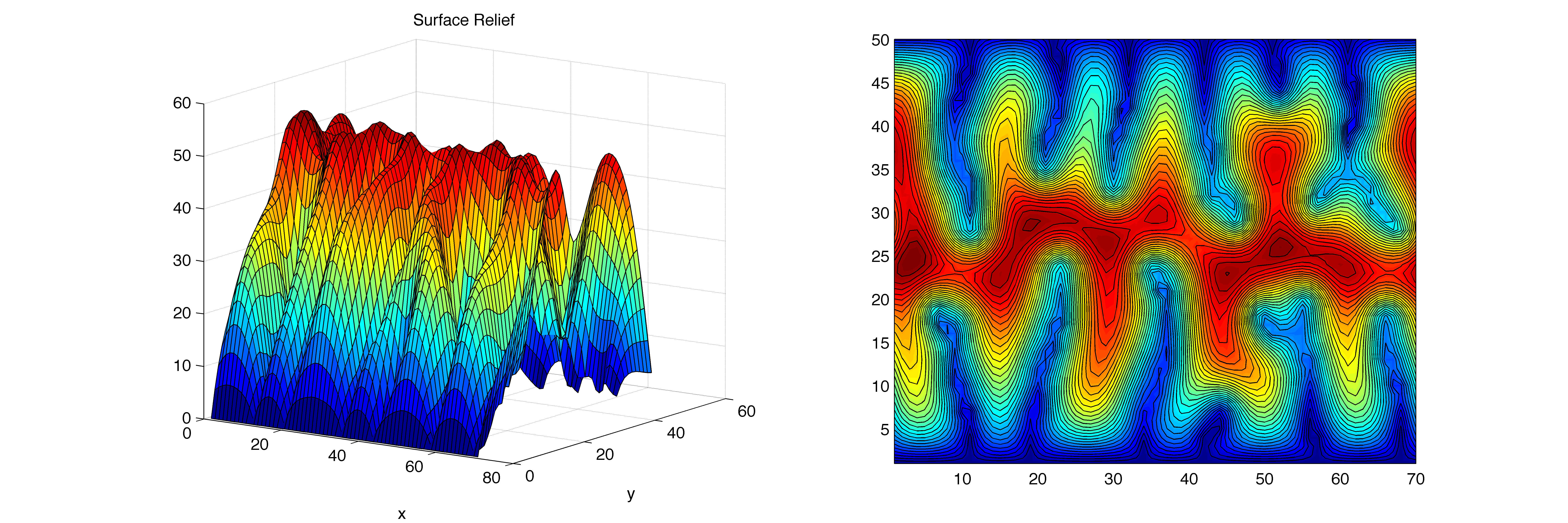Landscape evolution model (code written by former EPS109 student Jeff Prancevic)Three movies for different models are available: movie 1, movie 2, and movie 3.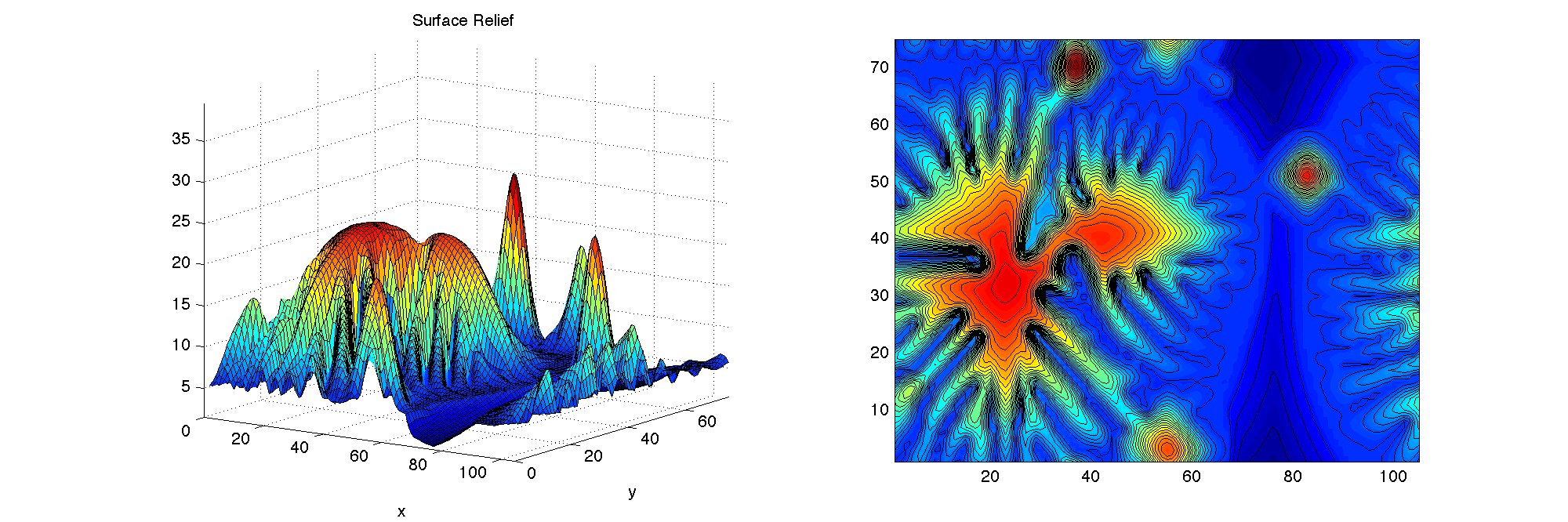A different landscape evolution model. Click on image to start the animation.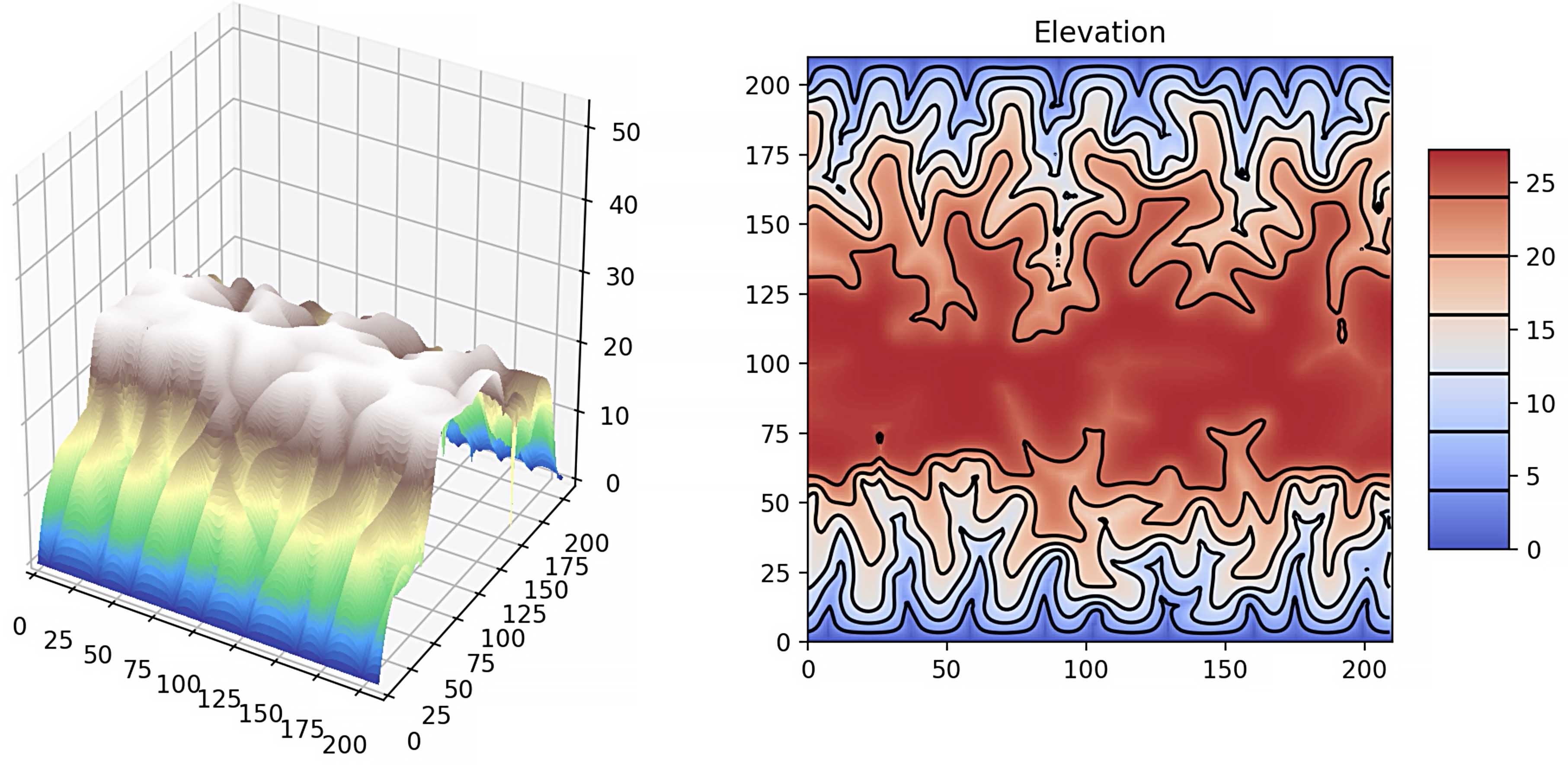Landscape evolution model rewritten in Python. Click on image to start the animation.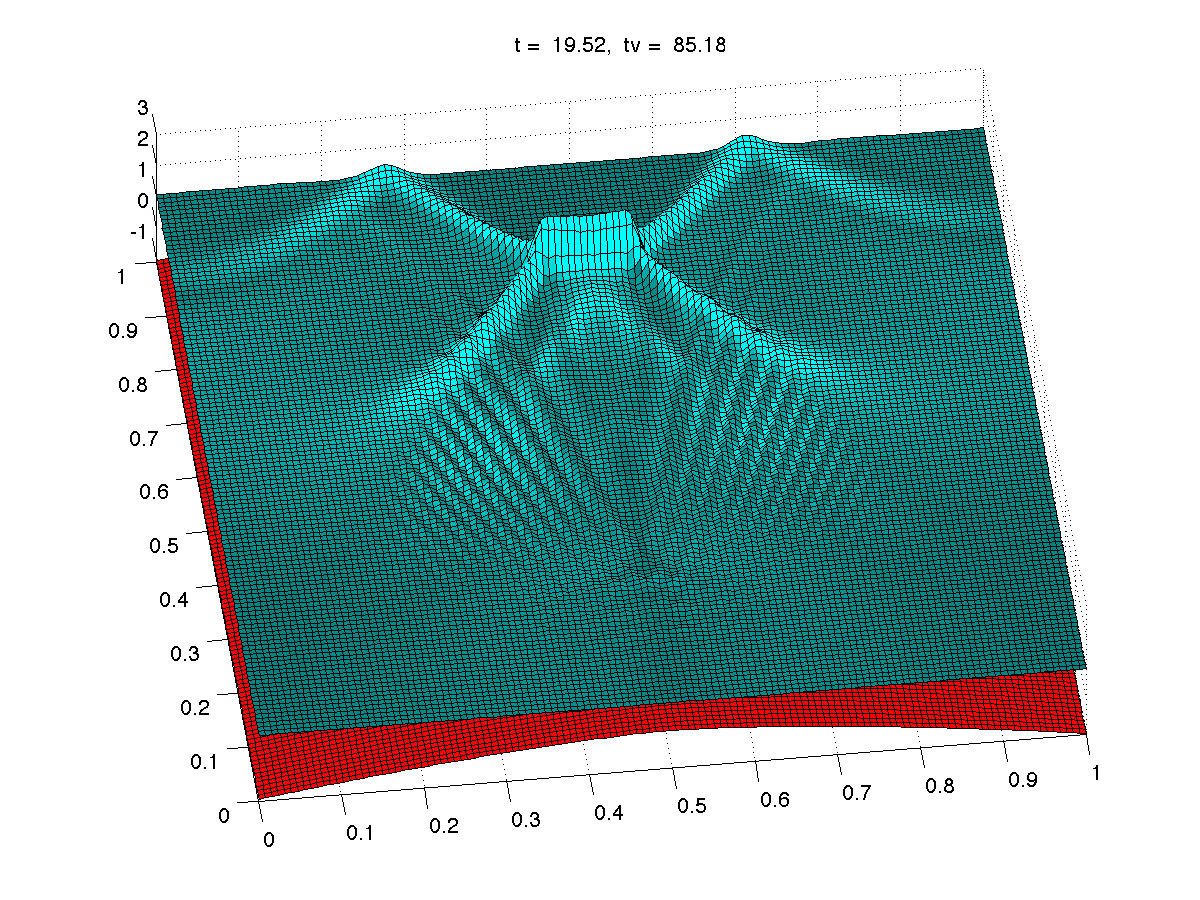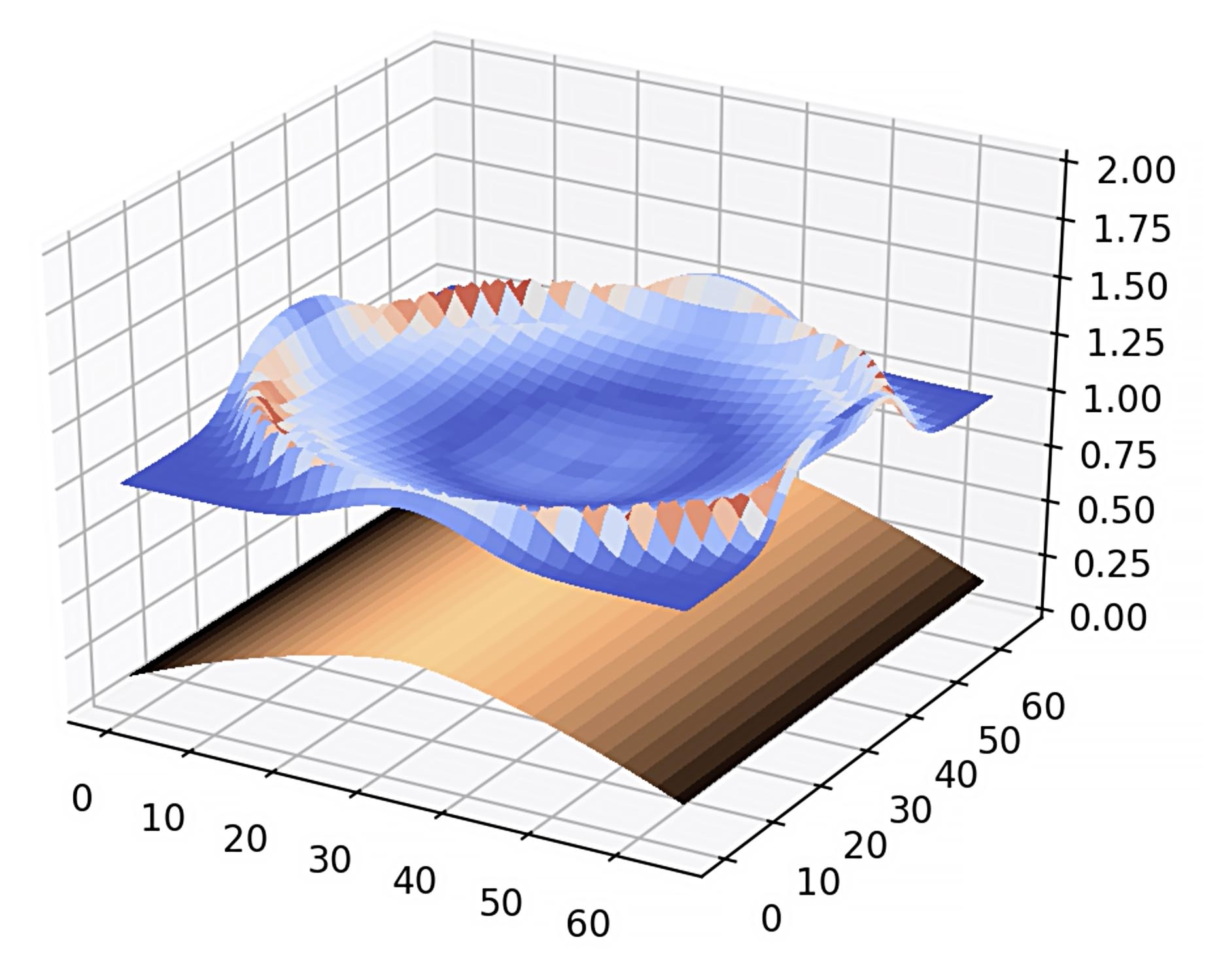Water wave traveling along a mid-ocean ridge. Numerical solution of the shallow water wave equation. Click on image to start animations 1 or 2 .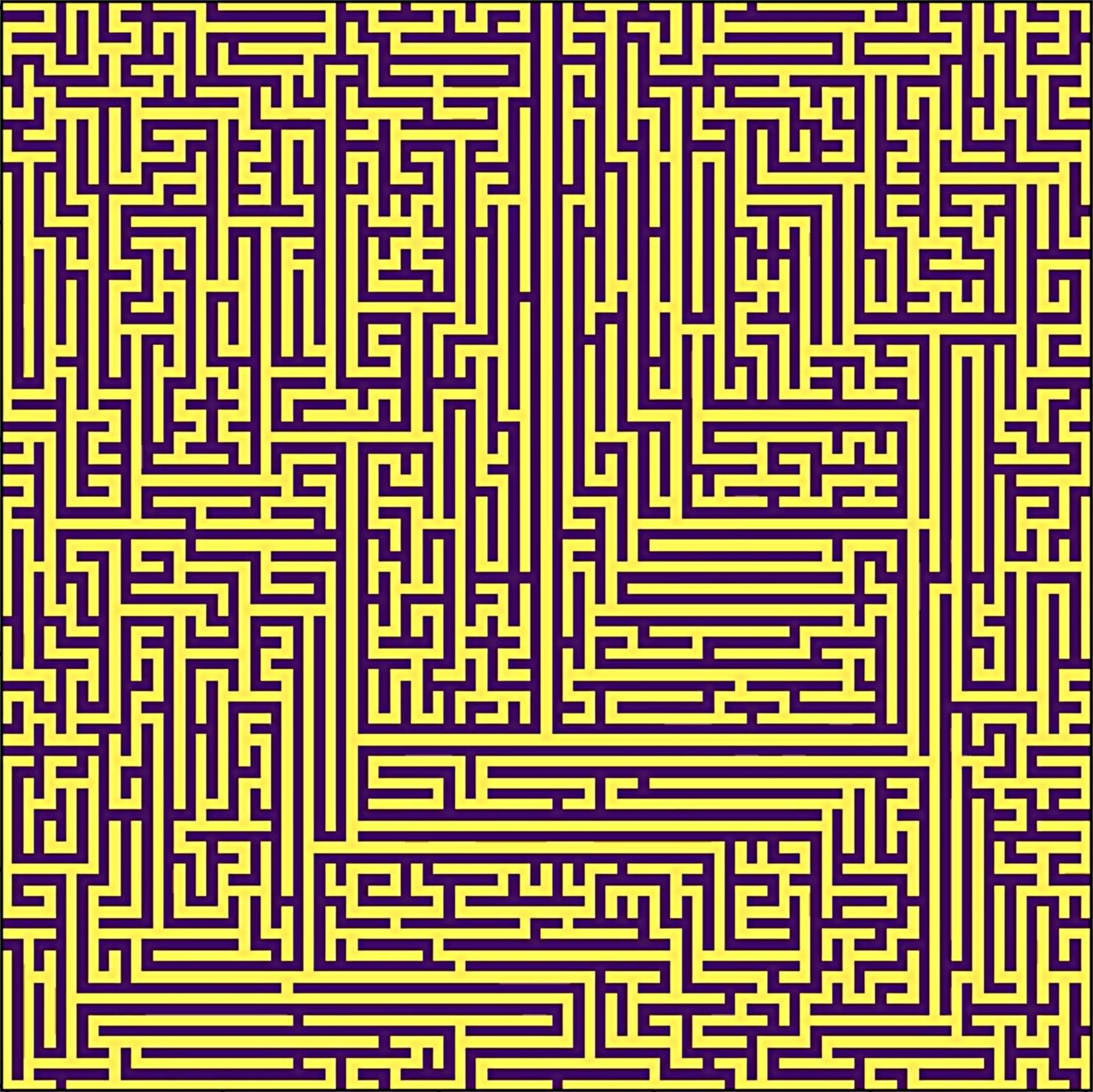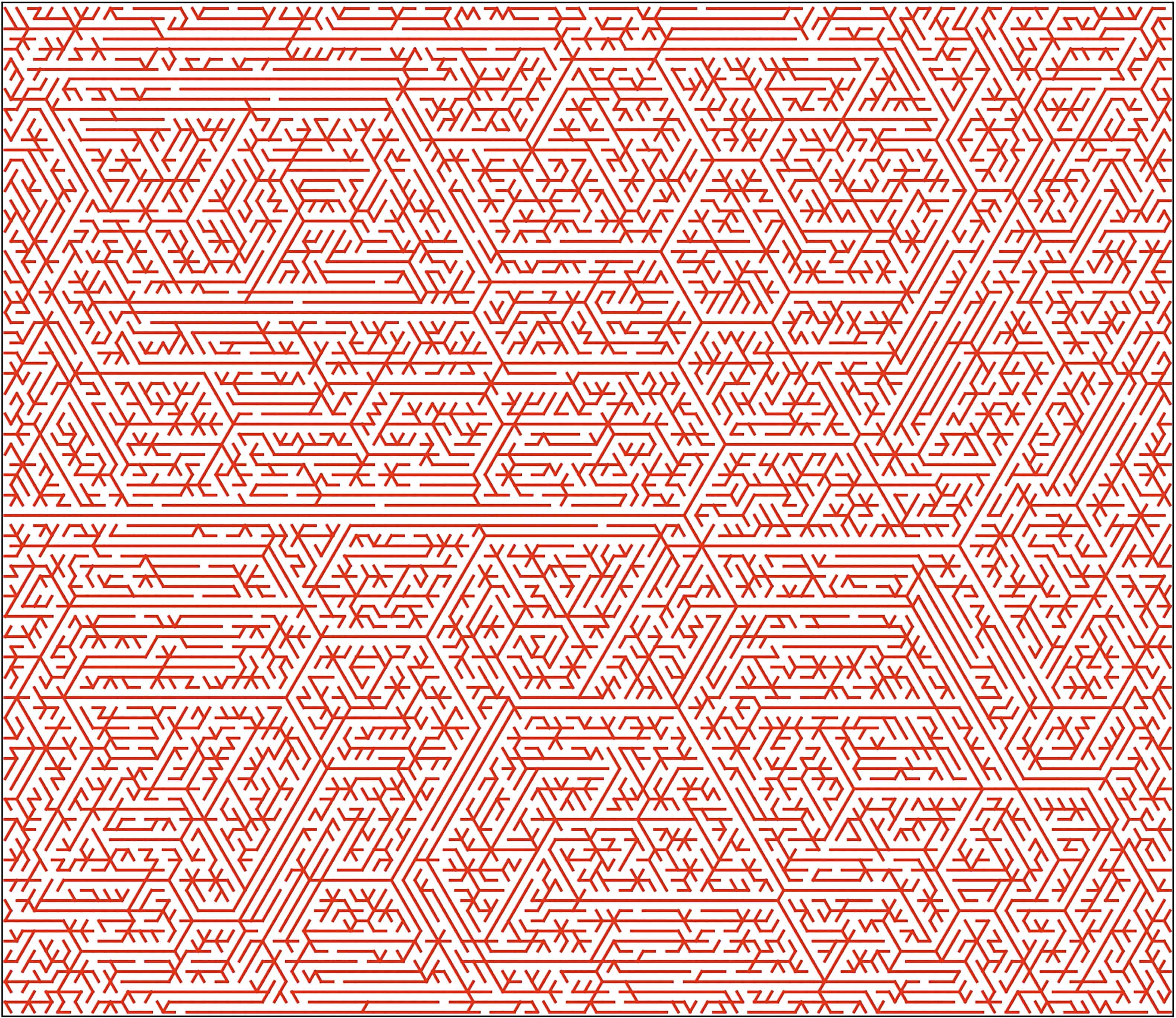Labyrinths were generated with 101 x 101, 201 x 202, and 401 x 401 resolution and also on a hexagonal grid.

Syllabus:

 Date Lecture topic Computer lab exercises Thu8/27 1: Introduction of course, historical overview, interplay of experiments, theory, and simulation Lab 1: Matlab as a pocket calculator Tue 9/1 2: Fractals in nature, models and computer simulations, fractal dimension and examples in topography and coast line structure Lab 2: Loops and plots in Matlab Tue9/3 3: Mandelbrot set and related fractals Tue 9/8 4: Random walks and diffusion limited aggregation (DLA), percolation theory Lab 3: Programming the Mandelbrot and Julia set Thu9/10 5: Group discussion on fractals Tue 9/15 6: Numerical algorithms for finding roots and minima Lab 4: DLA in 2D on a flat sheet Thu 9/17 7: Introduction to time independent partial differential equations (PDE), algorithms and stationary state of 1D heat equation Tue 9/22 8: Methods to find the stationary state of 2D heat equation Lab 5: Heat equation solver in 2D Thu 9/24 9: Time dependent PDEs, diffusion equation (heat and chemical diffusion), solution of the 1D heat equation, cooling of a lave dike Tue 9/29 10: Landscape erosion models Lab 6: Perron’s erosion model Thu 10/1 11: Wave equations and numerical solutions Tue 10/6 12: Shallow water wave equation, tsunamis Lab 7: 3D water wave simulations Thu 10/8 Midterm Tue 10/13 13: Introduction to ordinary differential equations, Euler’s method Lab 8: Keplerian orbits Thu 10/15 14: Newton’s law of motion, Keplerian orbits Tue 10/20 15: Planetary orbits, Hohmann transfers, spacecraft trajectories Lab 9: Lagrange points Thu 10/22 16: Runge-Kutta method Tue 10/27 17: Lagrange points, horseshoe and tadpole orbits of asteroids Lab 10: Exercise on tadpole and horseshoe orbits Thu 10/29 18: Strange attractors Tue 11/3 19: Chaotic dynamical systems and Lyaponov exponents Lab 11: Roessler attractor Thu 11/5 20: Simple models for climate change Tue 11/10 21: Molecular dynamics (MD) Lab 12: Box model for climate change Thu 11/12 22: Crystal structures, simulations in periodic boundary conditions Tue 11/17 23: Many particle simulations Lab 13: Molecular dynamics I Thu 11/19 24: Thermodynamic equilibrium, different states of matter Tue 11/24 25: Introduction to Monte Carlo (MC) simulations Lab 14: Molecular dynamics II Thu 11/26 Holiday Tue 12/1 26: Applications of MC simulations Lab 15: Comparison of MC and MD Thu 12/3 27: Curve fitting using the least-squares method, revisions, and exam preparations Tue 12/8 28: Students present their movie projects, part A Lab 16: Students present their movie projects, part B

The final grade will be calculated using the following percentages:

15% Grades for solving problem sets in weekly computer labs,
20% One midterm exam taken in class,
30% A final exam taken in class at the end of the semester.

Course description:

109. Computer simulations in Earth and Planetary Sciences (4) Two hours of lecture and two hours of computer lab exercises. Prerequisites: Math 1A or equivalent. Introduction to modern computer simulation methods and their application to selected Earth and Planetary Science problems. In hands-on computer labs, students will learn about numerical algorithms, learn to program and modify provided programs, and display the solution graphically. This is an introductory course and no programming experience is required. Examples include fractals in geophysics, properties of materials at high pressure, celestial mechanics, and diffusion processes in the Earth. Topics range from ordinary and partial differential equations to molecular dynamics and Monte Carlo simulations.

Motivation:

Computer simulations have become an integral part of earth and planetary science (EPS) but students arrive on campus with very different levels of computational skills. This course teaches the fundamental computational methods and their application in EPS. These skills are needed for later course work and research.

(*) C. B. Moler, “Numerical Computing with Matlab”, Society for Industrial and Apllied Mathematics (SIAM) Philadelphia, 2004, QA 297 M625 2004 ENGI, pages 14, 120, 187, 203, available online: http://www.mathworks.com/moler/index.html

Fractals:

(*) H.-O. Peitgen, D. Saupe (ed), The Science of Fractal Images”, Springer Verlag 1988, QA 614 .86 S351 1998 MATH, pages 40, 44, 50, 147, 155,

(*) D. L. Turcotte, “Fractals and Chaos in Geology and Geophysics”, Cambridge, U.K. ; New York : Cambridge University Press, 1997, page 14, 22,45, 59, 69, 71, 91, 97, 149, 201, 235, 299

(*) H.-O. Peigten, H. Jurgens, D. Saupe, “Fractals for the Classroom Part II Complex Systems and Mandelbort Set”, Springer 1992, QA 614 .86 P45 1992 v.2 MATH, pages 27. 58, 127, 199, 304, 318, 381, 401, 420, 446, 453.

(*) B. B. Mandelbrot, “The Fractal Geometry of Nature”, W.H. Freeman and Co. NY 1983,  QA 447 M357 1983 MATH, pages 30, 112,

D.J. Higham, N. J. Highham, “Matlab Guide”. Society for Industrial and Apllied Mathematics (SIAM) Philadelphia, 2000, QA 297 H5217 2000 ENGI, pages 14, 17, 90, , 132, 178, 221, 246.

A. Stanoyevitch, “Introduction to MATLAB with Numercial Preliminaries” Wiley 2004, TA 345 S75 2004 ENGI, pages 177.

J. Feder, “Fractals” Plenuym Press, NY, 1988, QA 447 F371 1998 MATH.

Ordinary Differential Equations, Molecular Dynamics, Solar System Dynamics:

J.H. Mathews, K.D. Fink, “Numerical Methods Using Matlab”, 4th edition, Pearson Education Inc., 2004, QA 297 M39 2004 ENGI, pgaes: 262, 418, 434, 465, 496, 505, 544, 557, 567,

(*) J. B. Marion, S. T. Thornton, “Classical Dynamics of particles and systems”, Saunders College Publishing, Fort Worth Philadelphia.

C.D. Murray, S.F Dermott, “Solar Systems Dynamics”, Cambridge University Press, 1999.

I. De Pater, J.J. Lissauer, “Planetary Science”, Cambridge University Press, 2001.

M. P. Allen, D.J. Tildesley, “Computer Simulations of Liquids”, Oxford University Press.

S. Nakamura, “Numerical Analysis and Graphic Visualization with Matlab” 2nd edition, Prentice Hall PTR 2001, T 385 N34 2002 ENGI, pages 63, 302, 385, 396, 453, 465.

W. J. Palm III, “Introduction to Matlb 6 for Engineers”, McGraw-Hill, 2001, TA 345 P35 2001 ENGI, pages  452.

H. B. Wilson, L. H Turcotte, “Advanced Mathematics and Mechanics Applications Using Matlab”, 2nd edition, CRC Press 1997, TA 354 W55 1997 ENGI, pages: 271, 352, 382.

A.H.Nayfeh, B. Balachandram, “Applied Nonlinear Dynamics, Analytical Computational, and Experimental Maethods”, Wiley, QA 845 N39 1994 ENGI.

E. A. Jackson, “Perspectives of Non-linear Dynamics”, Cambridge University Press, 1989, QA 845 J28 1989 V.1 ENGI

V.S. Anishchenko, V. Astakhov, A. Neiman, T. Vadivasova, L. Schimansky-Geier, “Nonlinear Dynamics of Chaotic and Stochastic Systems” Springer 2007, QA 845 .N667 2007 ENGI, pages 110.

Monte Carlo:

M. H. Kalos, P. A. Whitlock, “Monte Carlo Methods”, John Wiley & Sons, NY.

Partial Differential Equations:

(*) D. L. Turcotte and G. Schubert, “Geodynamics”, Cambridge ; New York : Cambridge University Press, 2002.

X.-S. Yang, “An Introduction to Computational Engineering with Matlab”, Cambridge International Science Publishing, 2006, TA 345 Y36 2006 ENGI, pages 33, 53, 57, 79, 224

W. E. Boyce, R. DiPirma, “Elementary Differential Equations and Boundary Value Problems”, Textbook, John Wiley & Sons, NY.

Climate:

K. Mcguffie, A. Henderson-Sellers, “A Climate modeling Primer”, Wiley 1997, QC 981 M482 1997 EART, page 85

(*) indicates the most relevant books.